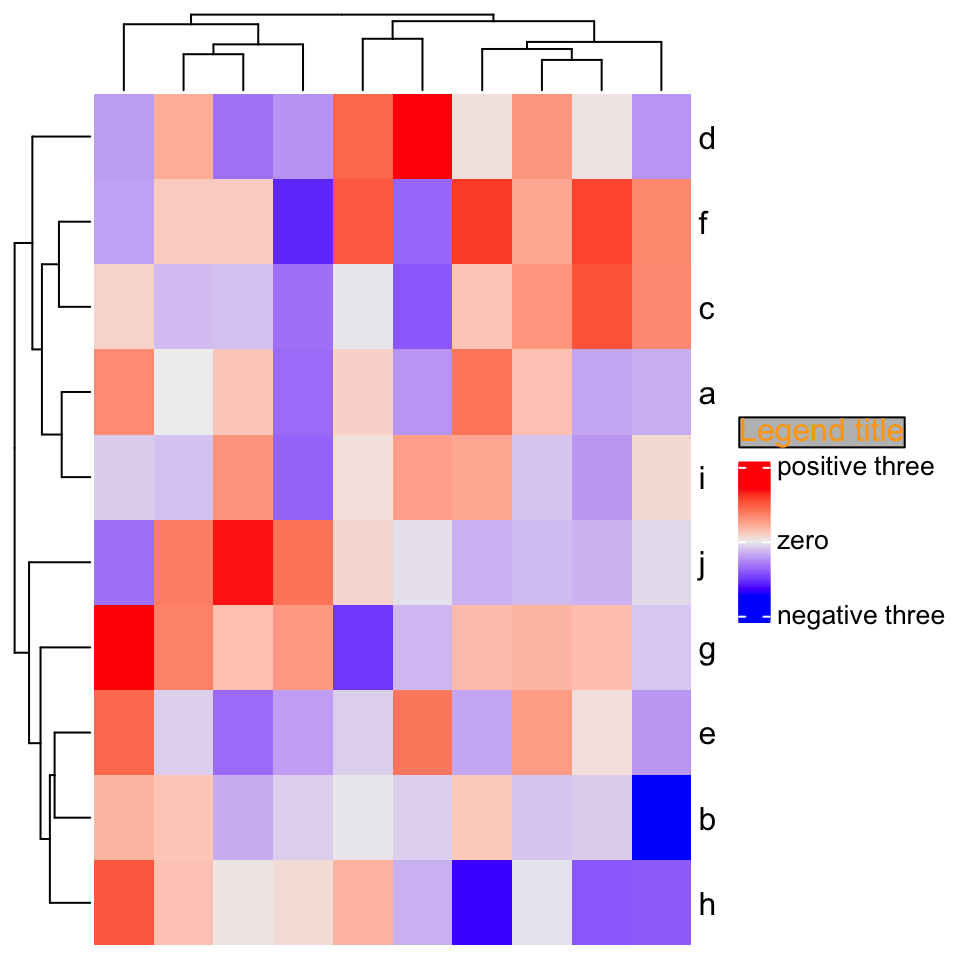# 10 Integrate with other packages

## 10.1 pheatmap

pheatmap is a great R package for making heatmaps, inspiring a lot of other heatmap packages such as ComplexHeatmap. From version 2.5.2 of ComplexHeatmap, I implemented a new ComplexHeatmap::pheatmap() function which actually maps all the parameters in pheatmap::pheatmap() to proper parameters in ComplexHeatmap::Heatmap(), which means, it converts a pheatmap to a complex heatmap. By doing this, the most significant improvement is now you can add multiple pheatmaps and annotations (defined by ComplexHeatmap::rowAnnotation()).

ComplexHeatmap::pheatmap() includes all arguments in pheatmap::pheatmap(), which means, you don’t need to do any adaptation on your pheatmap code, you just rerun your pheatmap code and it will automatically and nicely convert to the complex heatmap.

Some arguments in pheatmap::pheatmap() are disabled and ignored in this translation, listed as follows:

• kmeans_k
• filename
• width
• height
• silent

The usage of remaining arguments is exactly the same as in pheatmap::pheatmap().

In pheatmap::pheatmap(), the color argument is specified with a long color vector, e.g. :

pheatmap::pheatmap(mat,
color = colorRampPalette(rev(brewer.pal(n = 7, name = "RdYlBu")))(100)
)

You can use the same setting of color in ComplexHeatmap::pheatmap(), but you can also simplify it as:

ComplexHeatmap::pheatmap(mat,
color = rev(brewer.pal(n = 7, name = "RdYlBu"))
)

The colors for individual values are automatically interpolated.

### 10.1.1 Examples

First we load an example dataset which is from the “Examples” section of the documentation of pheatmap::pheatmap() function .

library(ComplexHeatmap)
test = matrix(rnorm(200), 20, 10)
test[1:10, seq(1, 10, 2)] = test[1:10, seq(1, 10, 2)] + 3
test[11:20, seq(2, 10, 2)] = test[11:20, seq(2, 10, 2)] + 2
test[15:20, seq(2, 10, 2)] = test[15:20, seq(2, 10, 2)] + 4
colnames(test) = paste("Test", 1:10, sep = "")
rownames(test) = paste("Gene", 1:20, sep = "")

Calling pheatmap() (which is now actually ComplexHeatmap::pheatmap()) generates a similar heatmap as by pheatmap::pheatmap().

pheatmap(test)  # this is ComplexHeatmap::pheatmap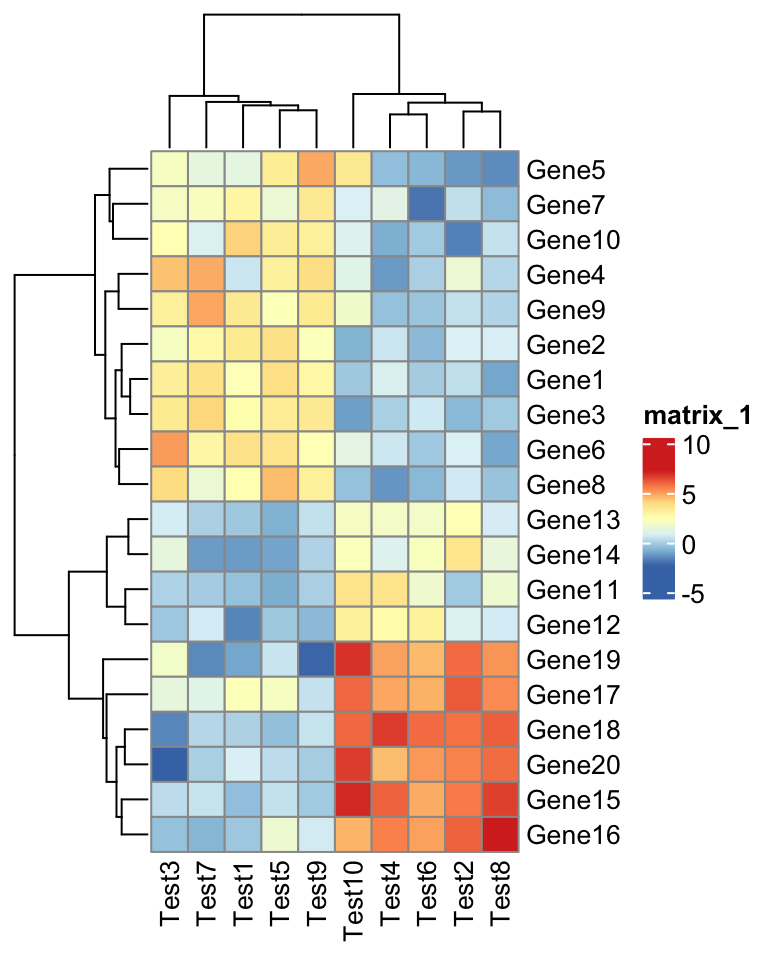Everything looks the same except the style of the heatmap legend. There are also some other visual difference which you can find in the “Comparisons” section in this post.

The next one is an example for setting annotations (you should be familiar with how to set these data frames and color list if you are a pheatmap user).

annotation_col = data.frame(
CellType = factor(rep(c("CT1", "CT2"), 5)),
Time = 1:5
)
rownames(annotation_col) = paste("Test", 1:10, sep = "")

annotation_row = data.frame(
GeneClass = factor(rep(c("Path1", "Path2", "Path3"), c(10, 4, 6)))
)
rownames(annotation_row) = paste("Gene", 1:20, sep = "")

ann_colors = list(
Time = c("white", "firebrick"),
CellType = c(CT1 = "#1B9E77", CT2 = "#D95F02"),
GeneClass = c(Path1 = "#7570B3", Path2 = "#E7298A", Path3 = "#66A61E")
)
pheatmap(test, annotation_col = annotation_col, annotation_row = annotation_row,
annotation_colors = ann_colors)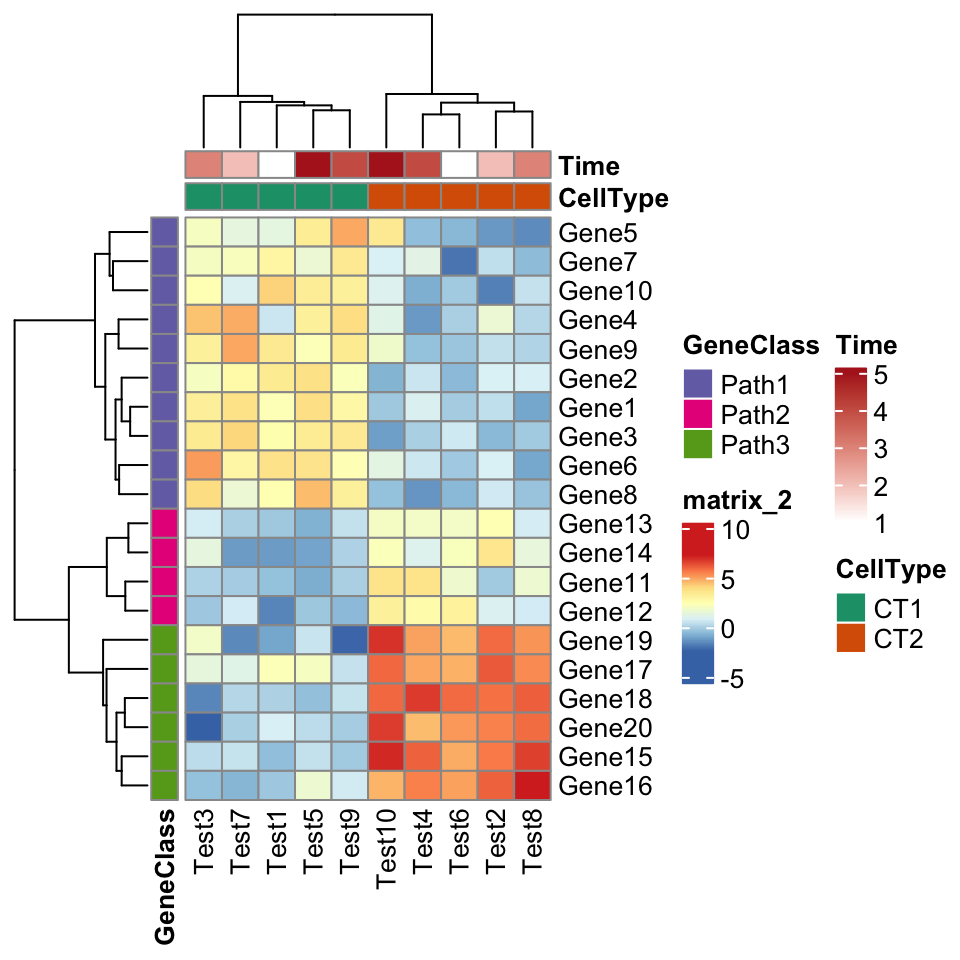ComplexHeatmap::pheatmap() returns a Heatmap object, so it can be added with other heatmaps and annotations. Or in other words, you can add multiple pheatmaps and annotations. Cool!

p1 = pheatmap(test, name = "mat1")
p2 = rowAnnotation(foo = anno_barplot(1:nrow(test)))
p3 = pheatmap(test, name = "mat2",
col = colorRampPalette(c("navy", "white", "firebrick3"))(50))
# or you can simply specify as
# p3 = pheatmap(test, name = "mat2", col = c("navy", "white", "firebrick3"))
p1 + p2 + p3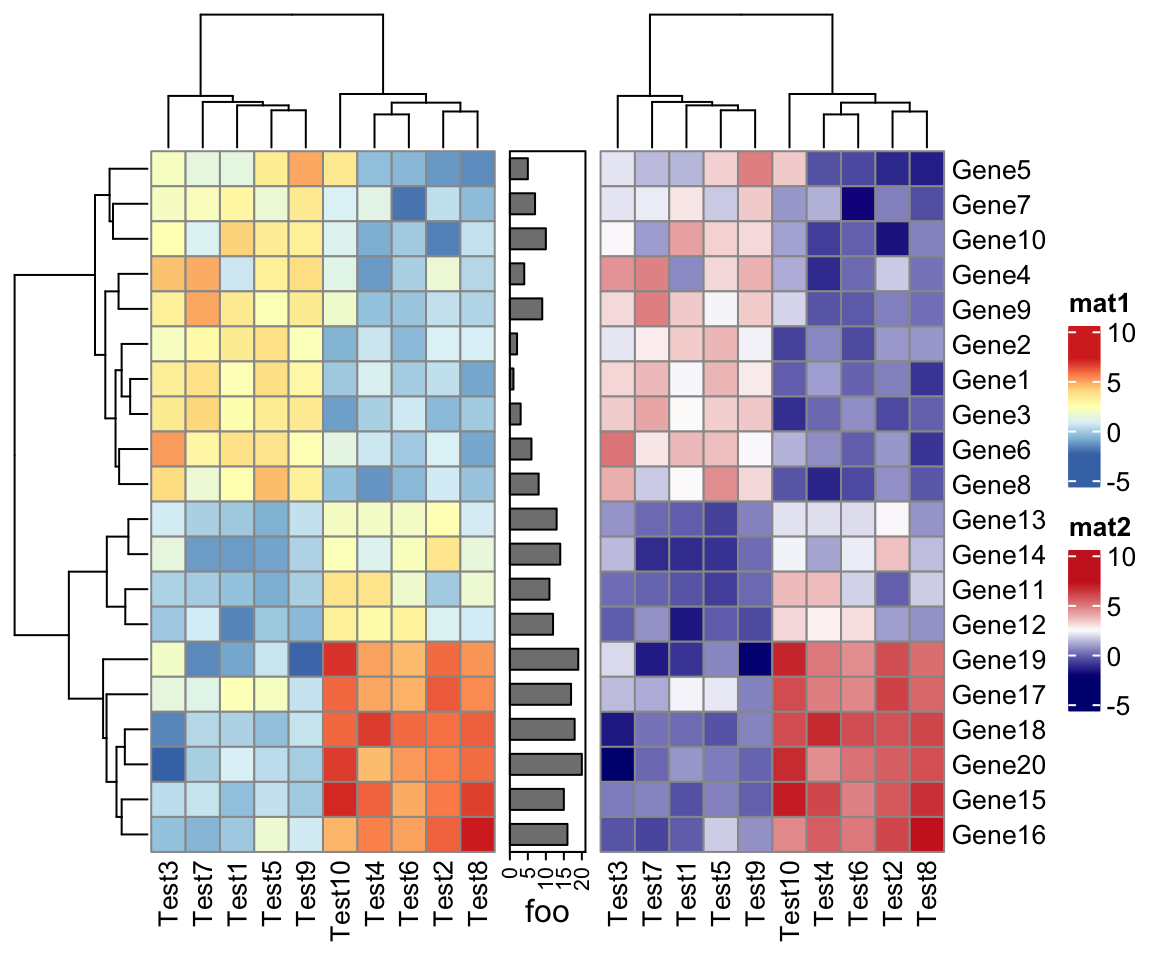Nevertheless, if you really want to add multiple pheatmaps, I still suggest you to directly use the Heatmap() function. You can find how to migrate from pheatmap::pheatmap() to ComplexHeatmap::Heatmap() in the next section.

In previous examples, the legend for row annotation is grouped with heatmap legend. This can be modified by setting legend_grouping argument in draw() function:

p = pheatmap(test, annotation_col = annotation_col, annotation_row = annotation_row,
annotation_colors = ann_colors)
draw(p, legend_grouping = "original")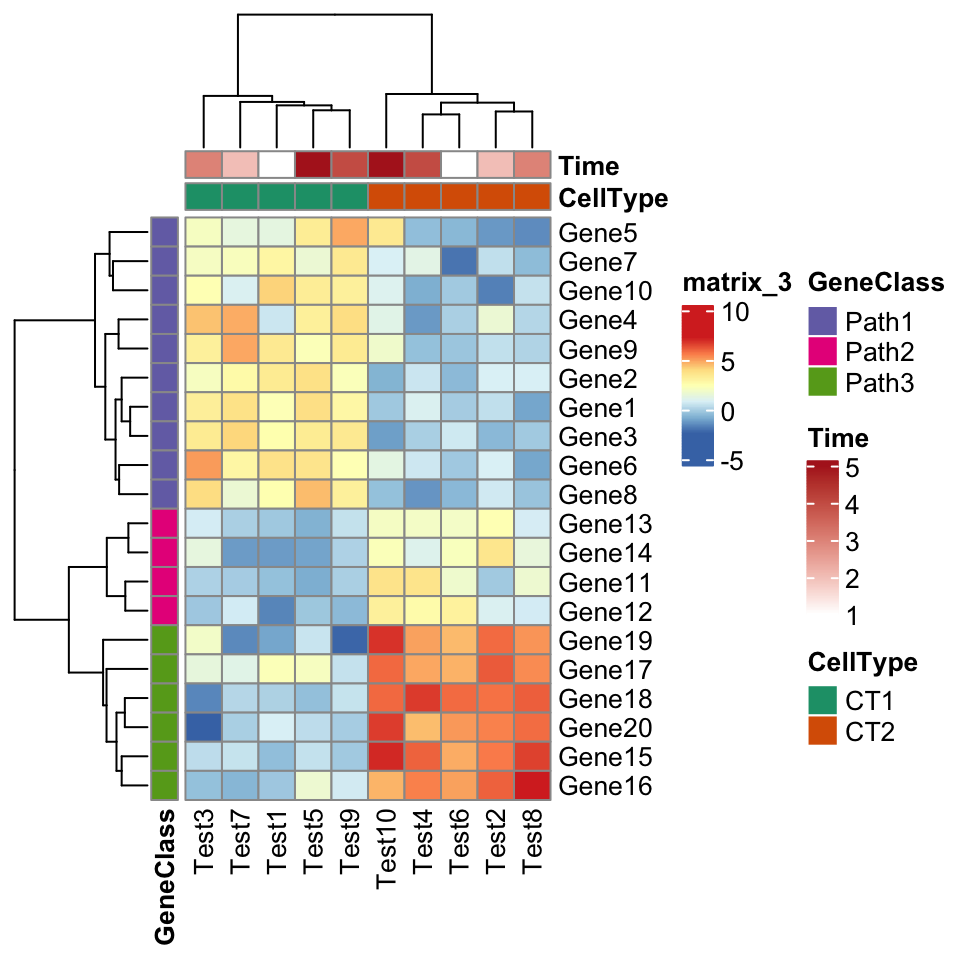One last thing is since ComplexHeatmap::pheatmap() returns a Heatmap object, if pheatmap() is not called in an interactive environment, e.g. in an R script, inside a function or in a for loop, you need to explicitly use draw() function:

for(...) {
p = pheatmap(...)
draw(p)
}

### 10.1.2 Translation

Following table lists how to map parameters in pheatmap::pheatmap() to ComplexHeatmap::Heatmap().

Arguments in pheatmap::pheatmap() Identical settings/arguments in ComplexHeatmap::Heatmap()
mat matrix
color Users can specify a color mapping function by circlize::colorRamp2(), or provide a vector of colors on which colors for individual values are linearly interpolated.
kmeans_k No corresponding parameter because it changes the matrix for heatmap.
breaks It should be specified in the color mapping function.
border_color rect_gp = gpar(col = border_color). In the annotations, it is HeatmapAnnotation(..., gp = gpar(col = border_color)).
cellwidth width = ncol(mat)*unit(cellwidth, "pt")
cellheight height = nrow(mat)*unit(cellheight, "pt")
scale Users should simply apply scale() on the matrix before sending to Heatmap().
cluster_rows cluster_rows
cluster_cols cluster_columns
clustering_distance_rows clustering_distance_rows. The value correlation should be changed to pearson.
clustering_distance_cols clustering_distance_columns, The value correlation should be changed to pearson.
clustering_method clustering_method_rows/clustering_method_columns
clustering_callback The processing on the dendrogram should be applied before sending to Heatmap().
cutree_rows row_split and row clustering should be applied.
cutree_cols column_split and column clustering should be applied.
treeheight_row row_dend_width = unit(treeheight_row, "pt")
treeheight_col column_dend_height = unit(treeheight_col, "pt")
legend show_heatmap_legend
legend_breaks heatmap_legend_param = list(at = legend_breaks)
legend_labels heatmap_legend_param = list(labels = legend_labels)
annotation_row left_annotatioin = rowAnnotation(df = annotation_row)
annotation_col top_annotation = HeatmapAnnotation(df = annotation_col)
annotation Not supported.
annotation_colors col argument in HeatmapAnnotation()/rowAnnotation().
annotation_legend show_legend argument in HeatmapAnnotation()/rowAnnotation().
annotation_names_row show_annotation_name in rowAnnotation().
annotation_names_col show_annotation_name in HeatmaoAnnotation().
drop_levels Unused levels are all dropped.
show_rownames show_row_names
show_colnames show_column_names
main column_title
fontsize gpar(fontsize = fontsize) in corresponding heatmap components.
fontsize_row row_names_gp = gpar(fontsize = fontsize_row)
fontsize_col column_names_gp = gpar(fontsize = fontsize_col)
angle_col column_names_rot. The rotation of row annotation names are not supported.
display_numbers Users should set a proper cell_fun or layer_fun (vectorized and faster version of cell_fun). E.g. if display_numbers is TRUE, layer_fun can be set as function(j, i, x, y, w, h, fill) { grid.text(sprintf(number_format, pindex(mat, i, j)), x = x, y = y, gp = gpar(col = number_color, fontsize = fontsize_number)) }. If display_numbers is a matrix, replace mat to display_numbers in the layer_fun.
number_format See above.
number_color See above.
fontsize_number See above.
gaps_row Users should construct a “splitting variable” and send to row_split. E.g. slices = diff(c(0, gaps_row, nrow(mat))); rep(seq_along(slices), times = slices).
gaps_col Users should construct a “splitting variable” and send to column_split.
labels_row row_labels
labels_col column_labels
filename No corresponding setting in Heatmap(). Users need to explicitly use e.g. pdf().
width No corresponding setting in Heatmap().
height No corresponding setting in Heatmap().
silent No corresponding setting in Heatmap().
na_col na_col

### 10.1.3 Comparisons

I ran all the example code in the “Examples” section of the documentation of pheatmap::pheatmap() function . I also implemented a wrapper function ComplexHeatmap::compare_pheatmap() which basically uses the same set of arguments for pheatmap::pheatmap() and ComplexHeatmap::pheatmap() and draws two heatmaps, so that you can directly see the similarity and difference of the two heatmap implementations.

compare_pheatmap(test)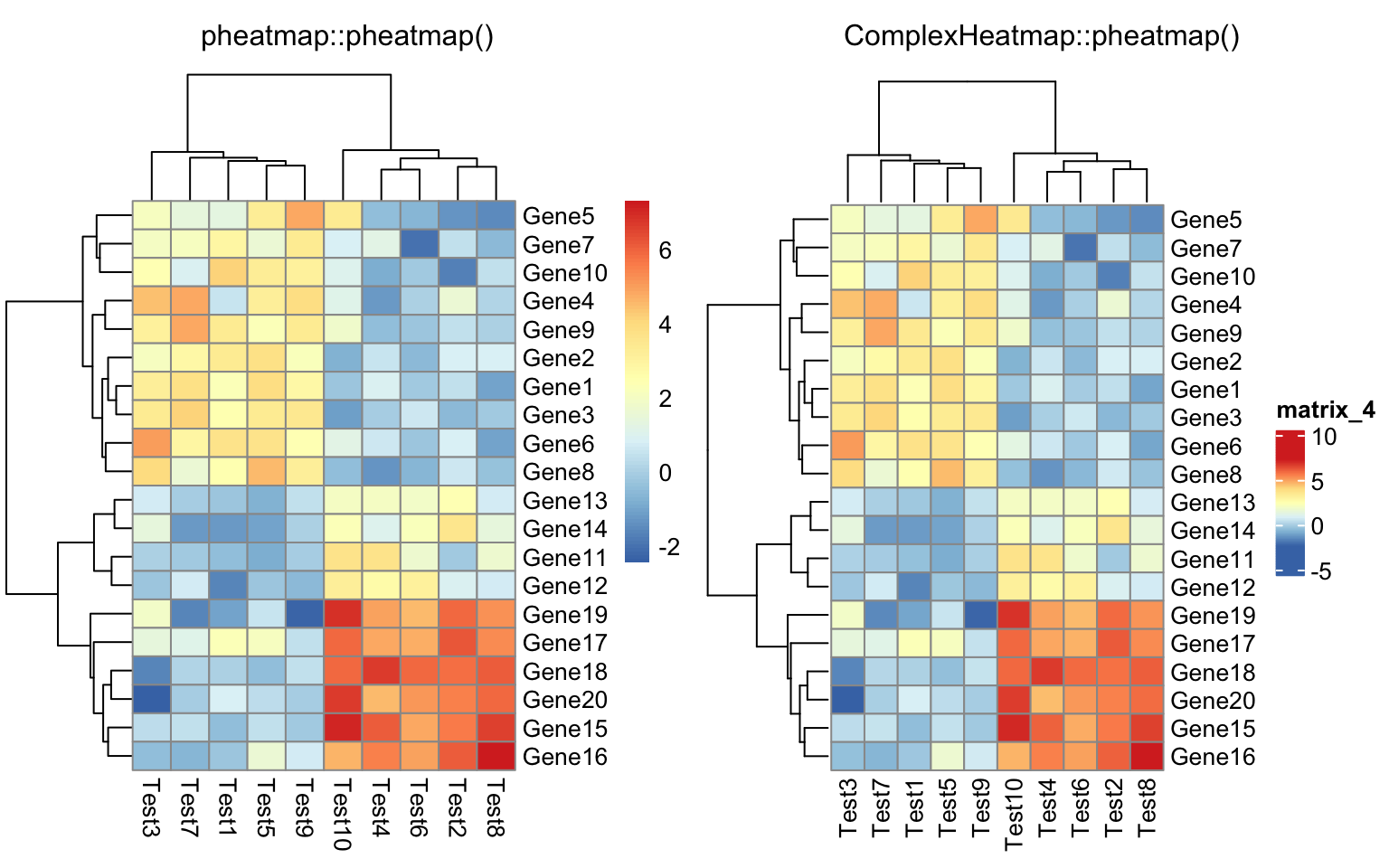compare_pheatmap(test, scale = "row", clustering_distance_rows = "correlation")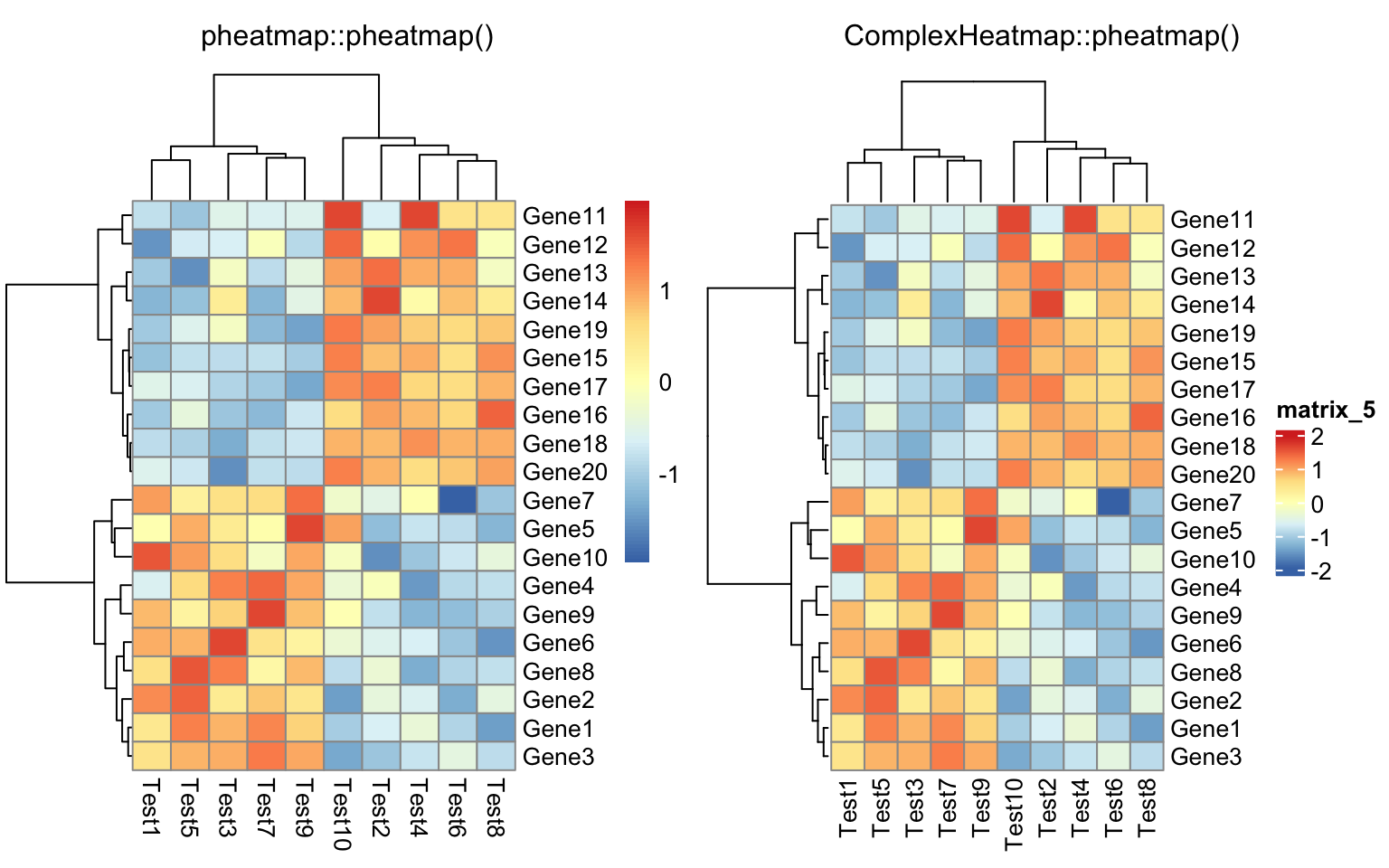compare_pheatmap(test,
color = colorRampPalette(c("navy", "white", "firebrick3"))(50))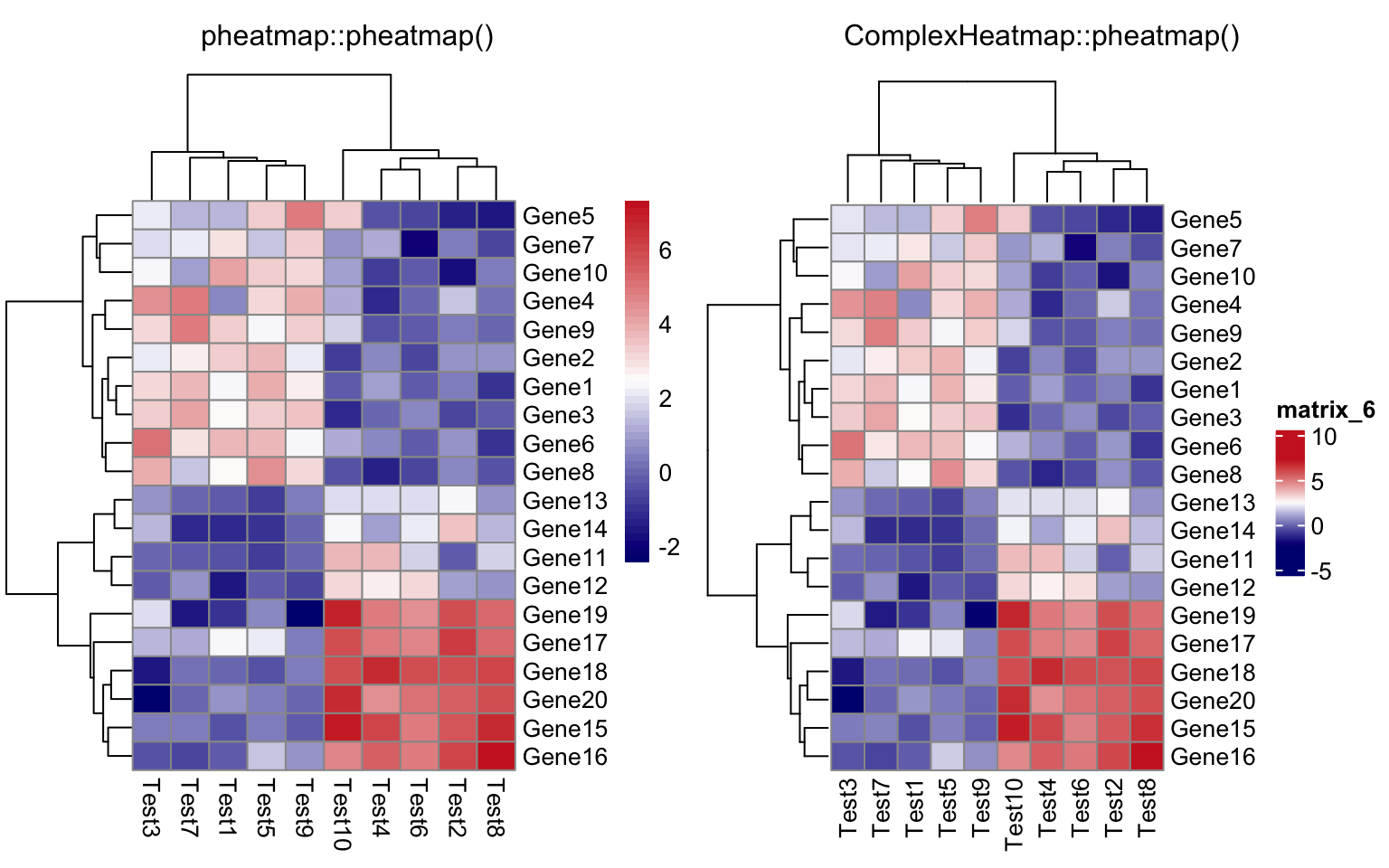compare_pheatmap(test, cluster_row = FALSE)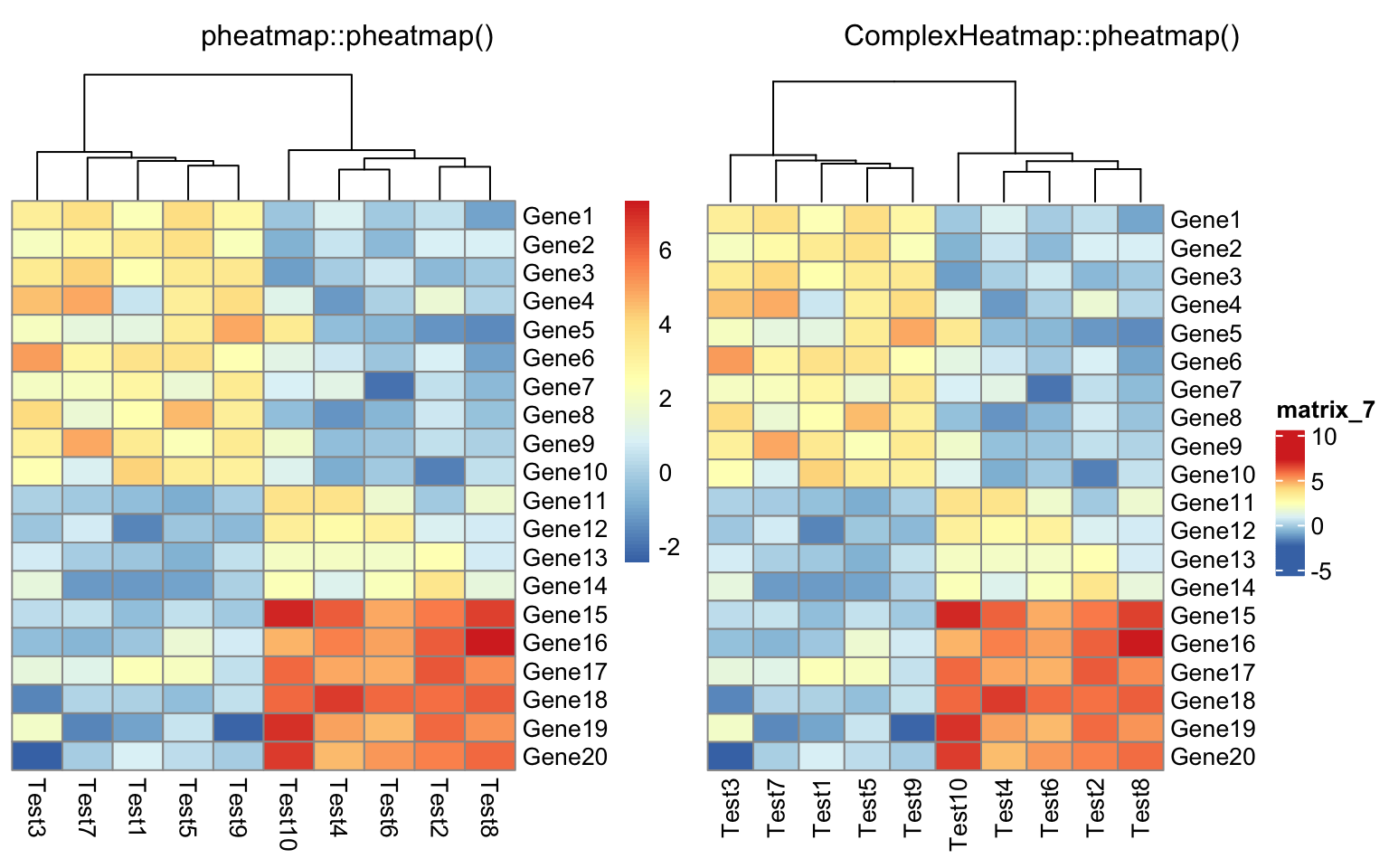compare_pheatmap(test, legend = FALSE)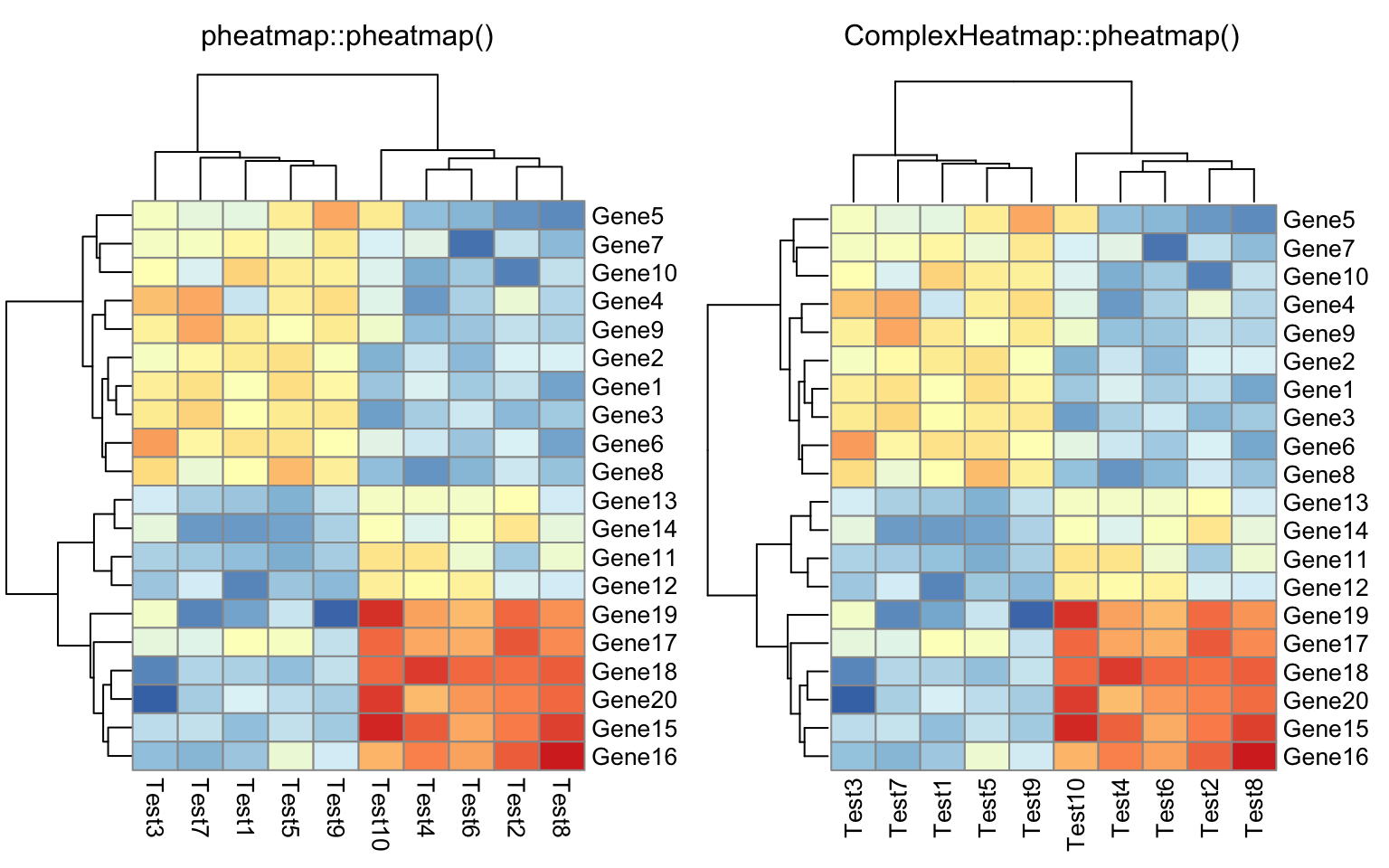compare_pheatmap(test, display_numbers = TRUE)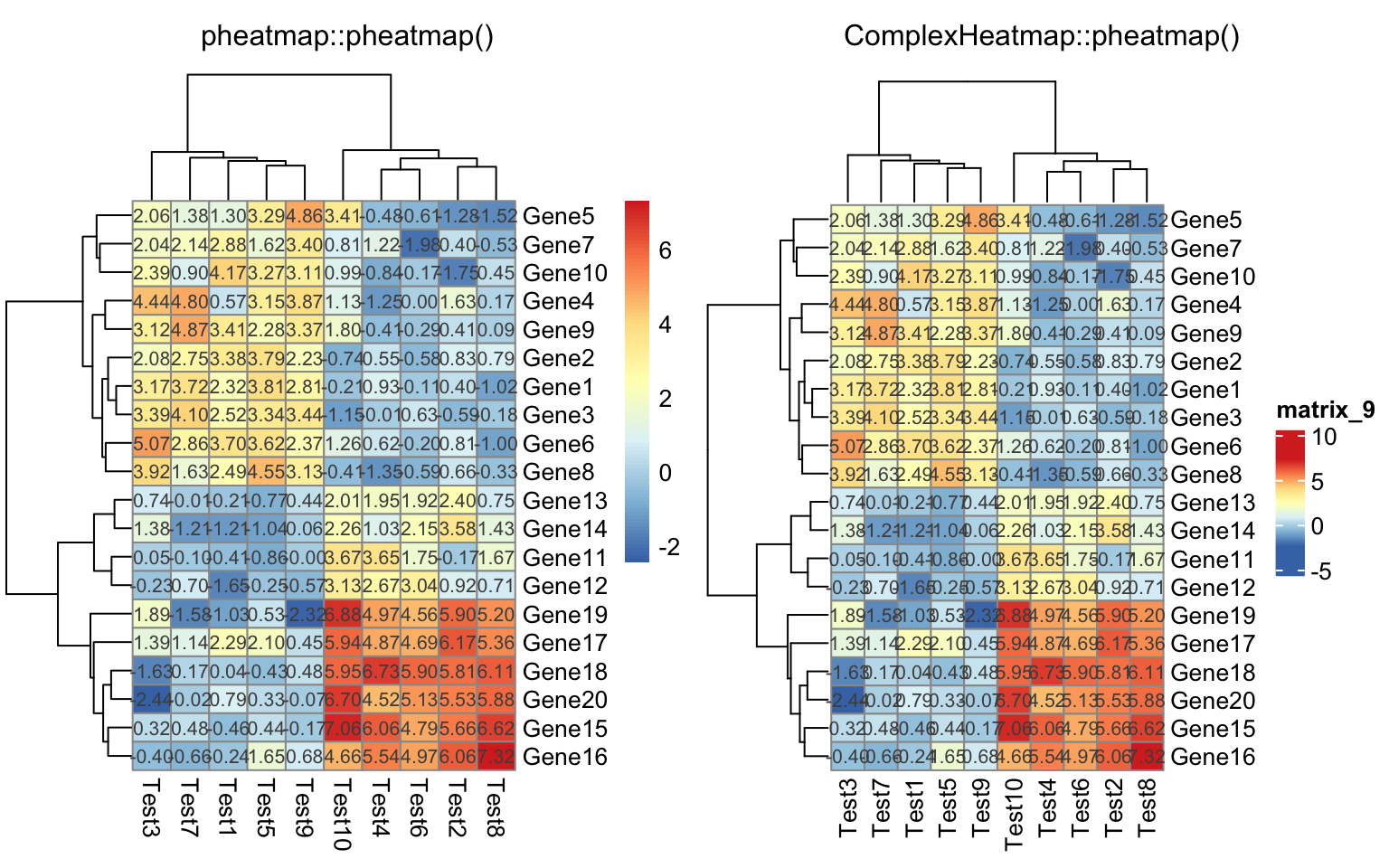compare_pheatmap(test, display_numbers = TRUE, number_format = "%.1e")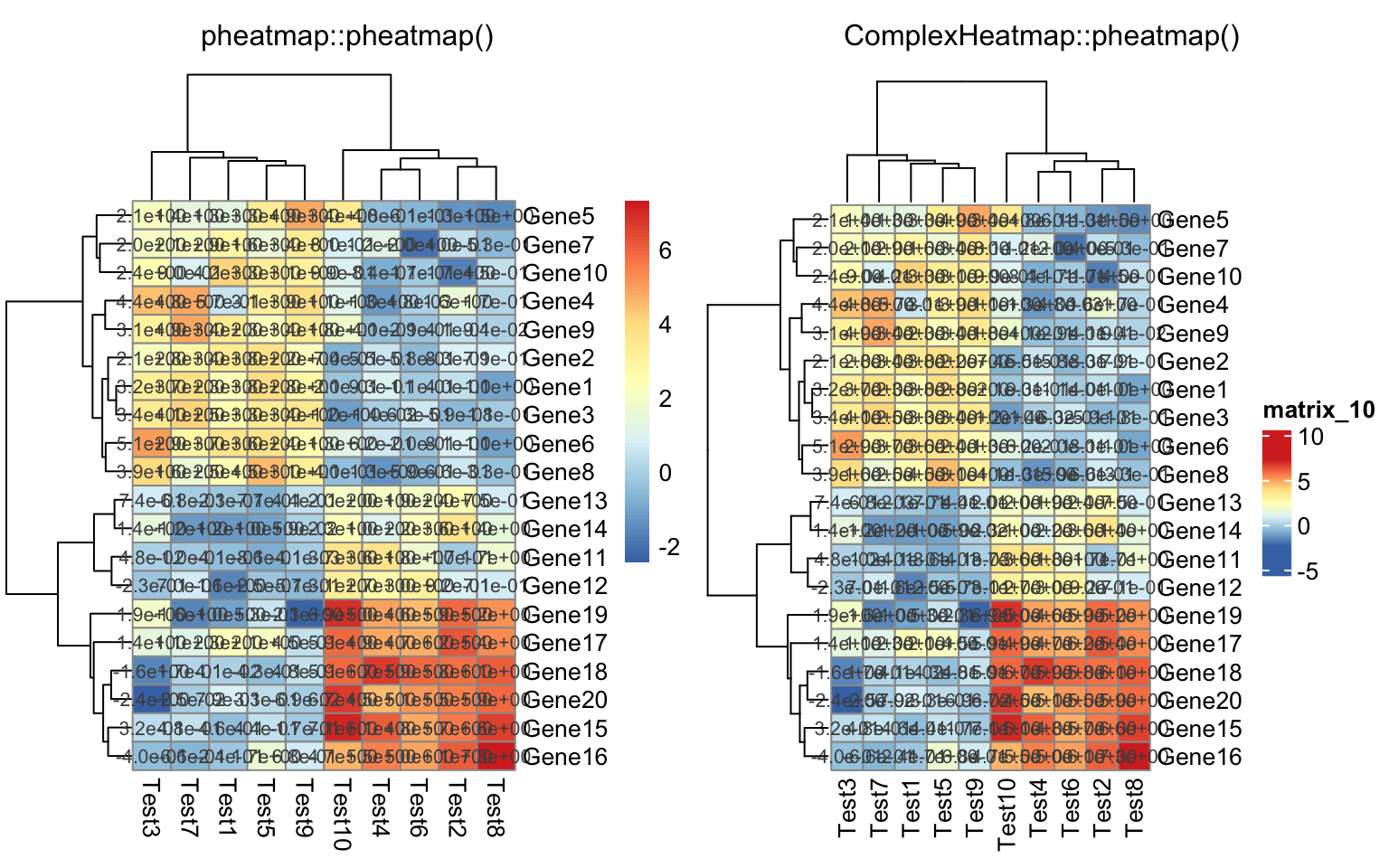compare_pheatmap(test,
display_numbers = matrix(ifelse(test > 5, "*", ""), nrow(test)))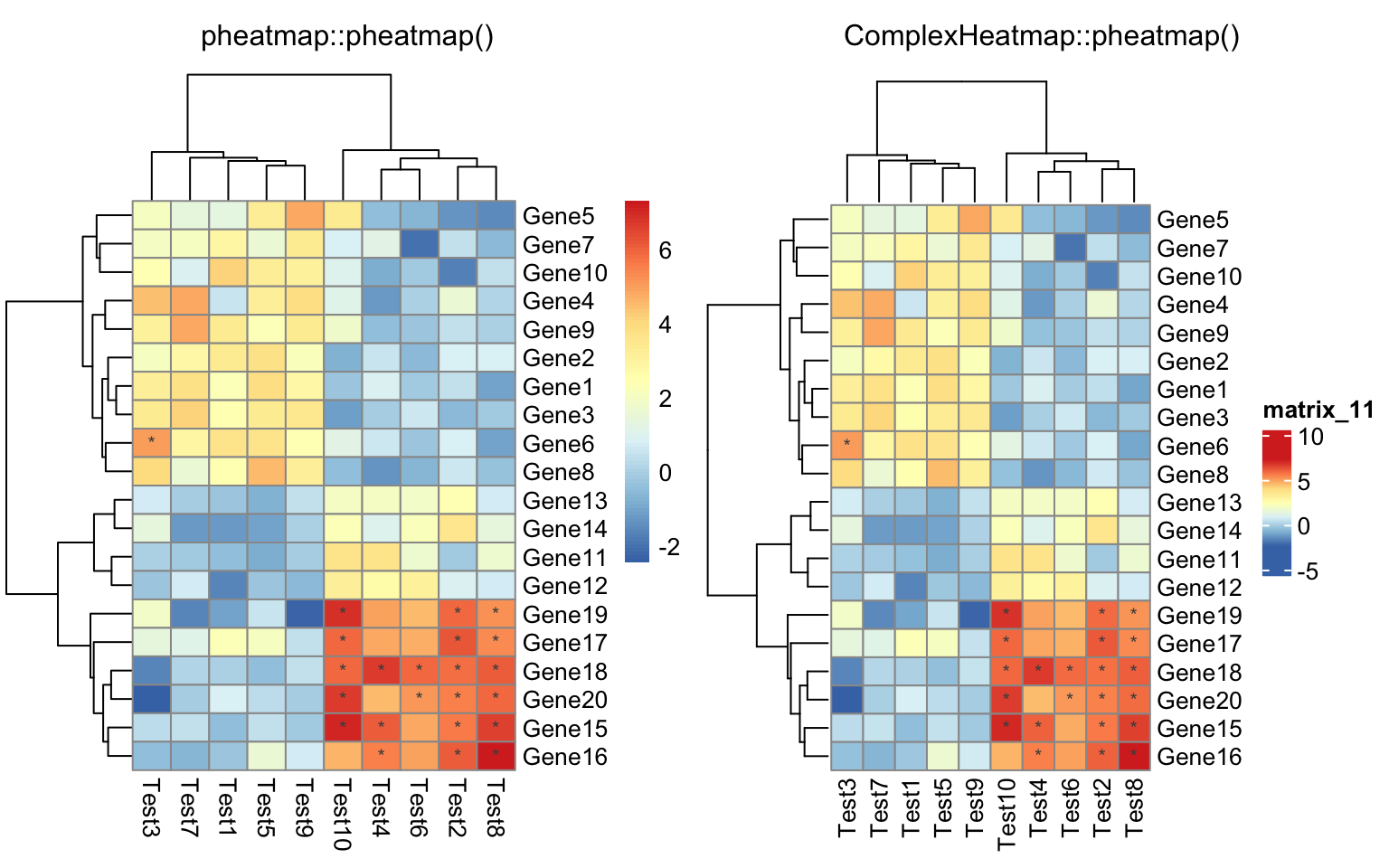compare_pheatmap(test, cluster_row = FALSE, legend_breaks = -1:4,
legend_labels = c("0", "1e-4", "1e-3", "1e-2", "1e-1", "1"))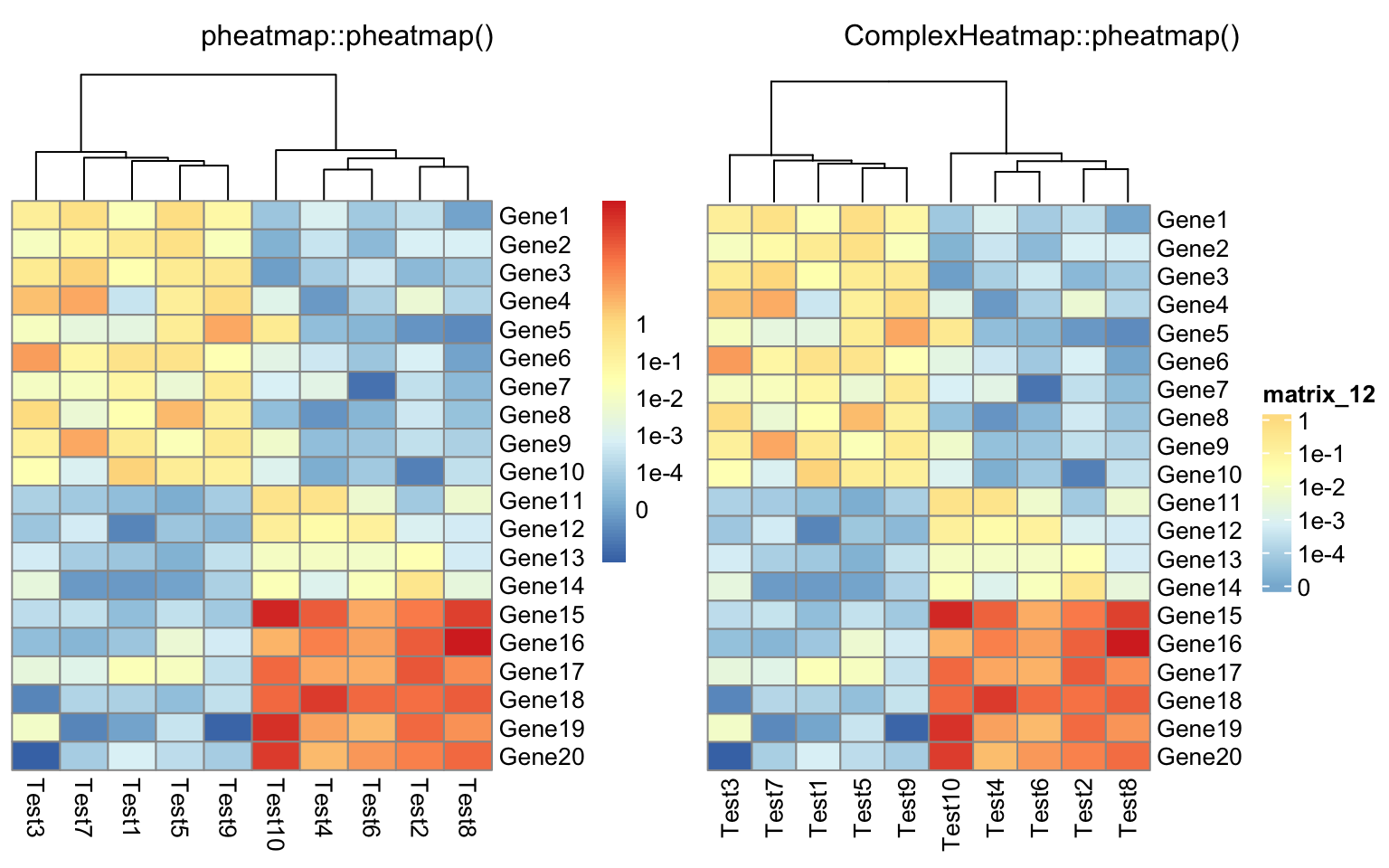compare_pheatmap(test, cellwidth = 15, cellheight = 12, main = "Example heatmap")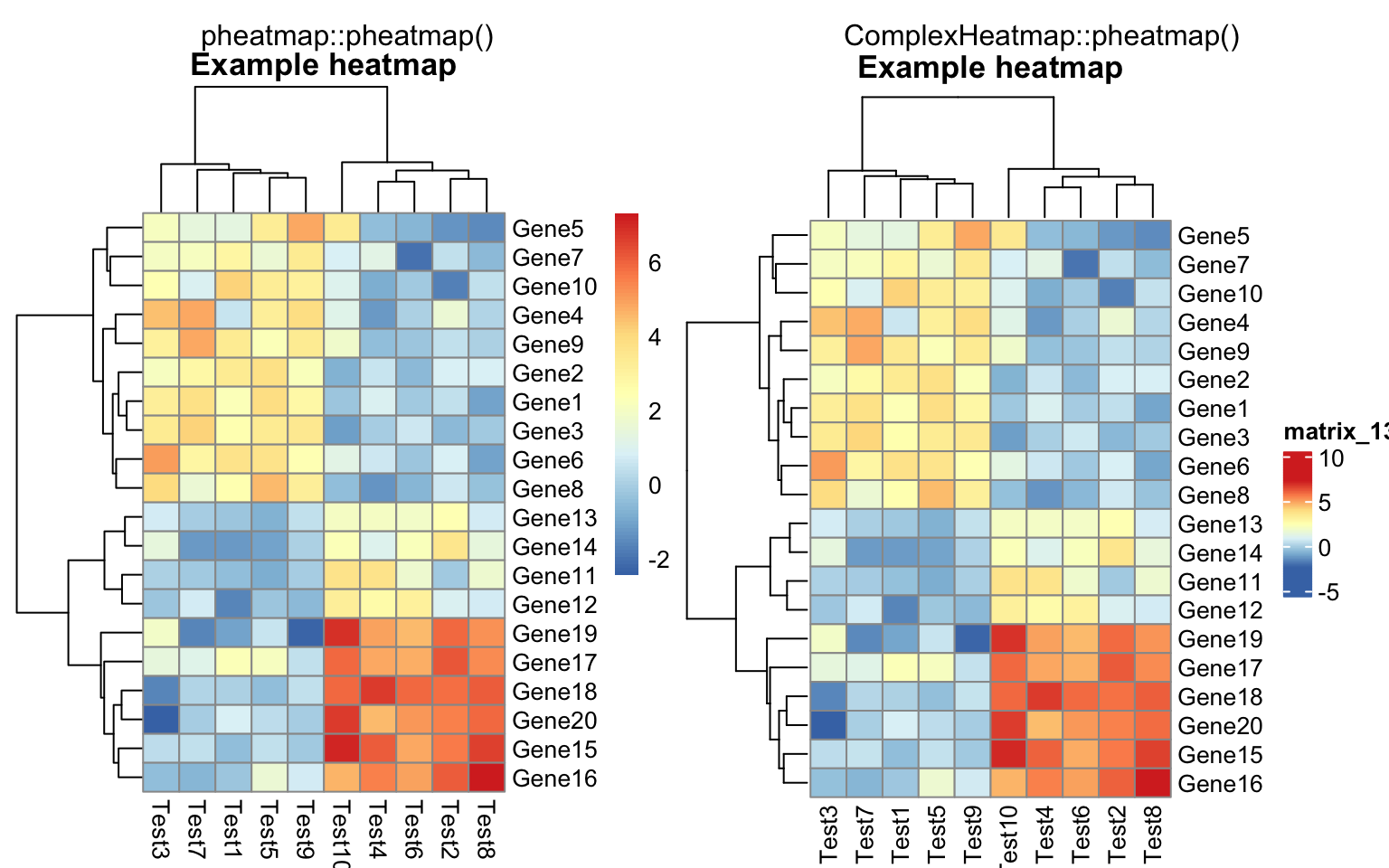annotation_col = data.frame(
CellType = factor(rep(c("CT1", "CT2"), 5)),
Time = 1:5
)
rownames(annotation_col) = paste("Test", 1:10, sep = "")

annotation_row = data.frame(
GeneClass = factor(rep(c("Path1", "Path2", "Path3"), c(10, 4, 6)))
)
rownames(annotation_row) = paste("Gene", 1:20, sep = "")

compare_pheatmap(test, annotation_col = annotation_col)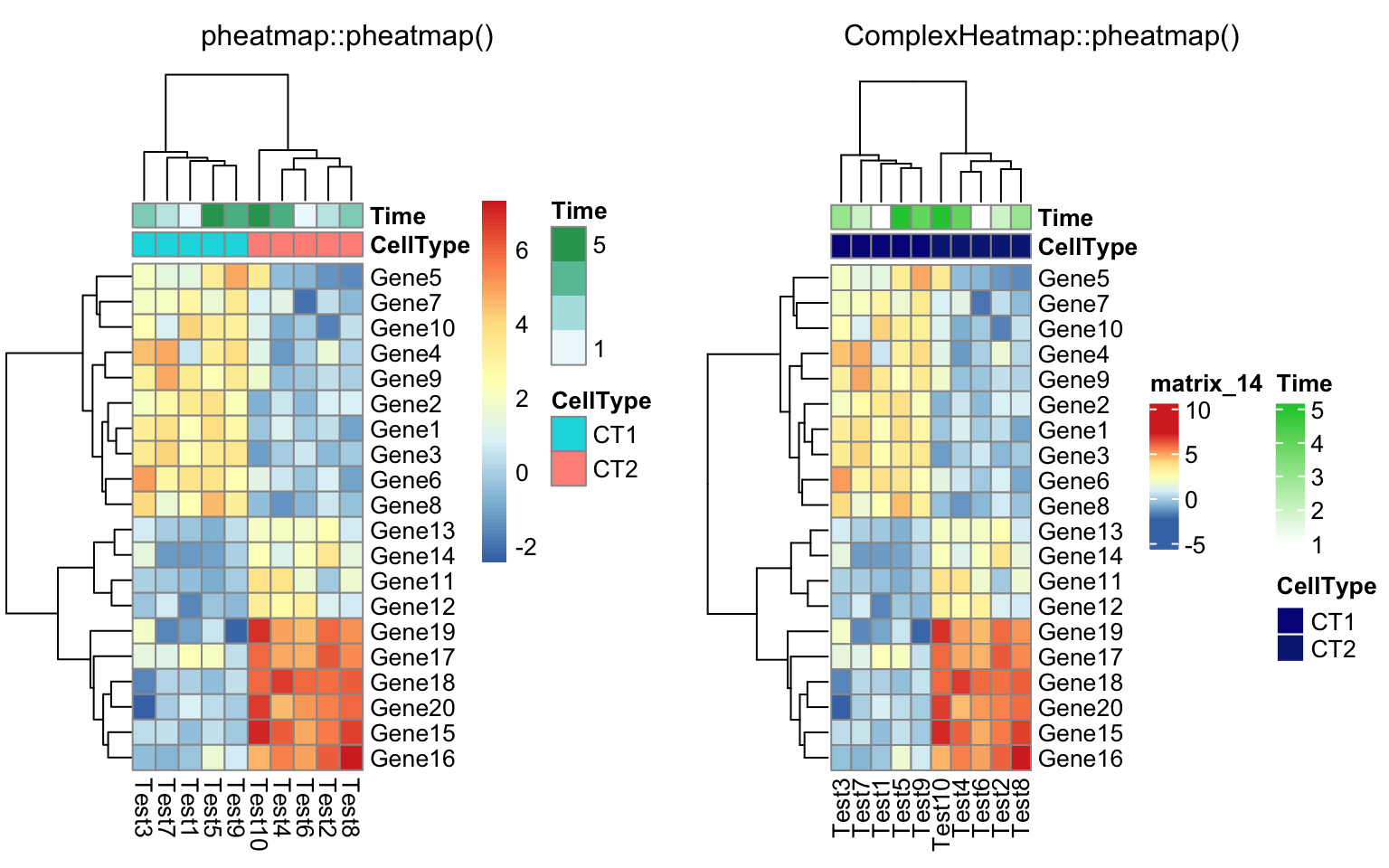compare_pheatmap(test, annotation_col = annotation_col, annotation_legend = FALSE)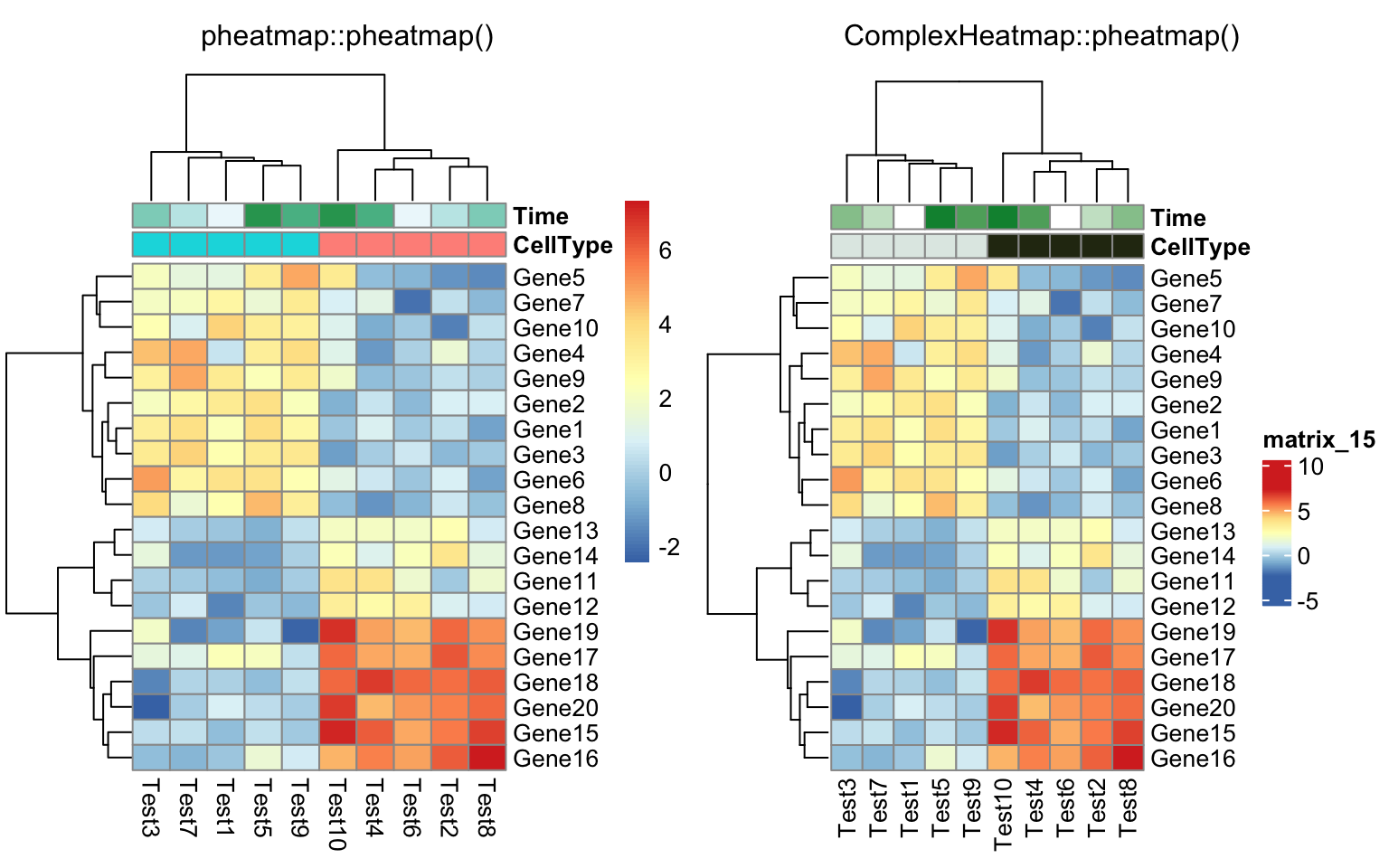compare_pheatmap(test, annotation_col = annotation_col,
annotation_row = annotation_row)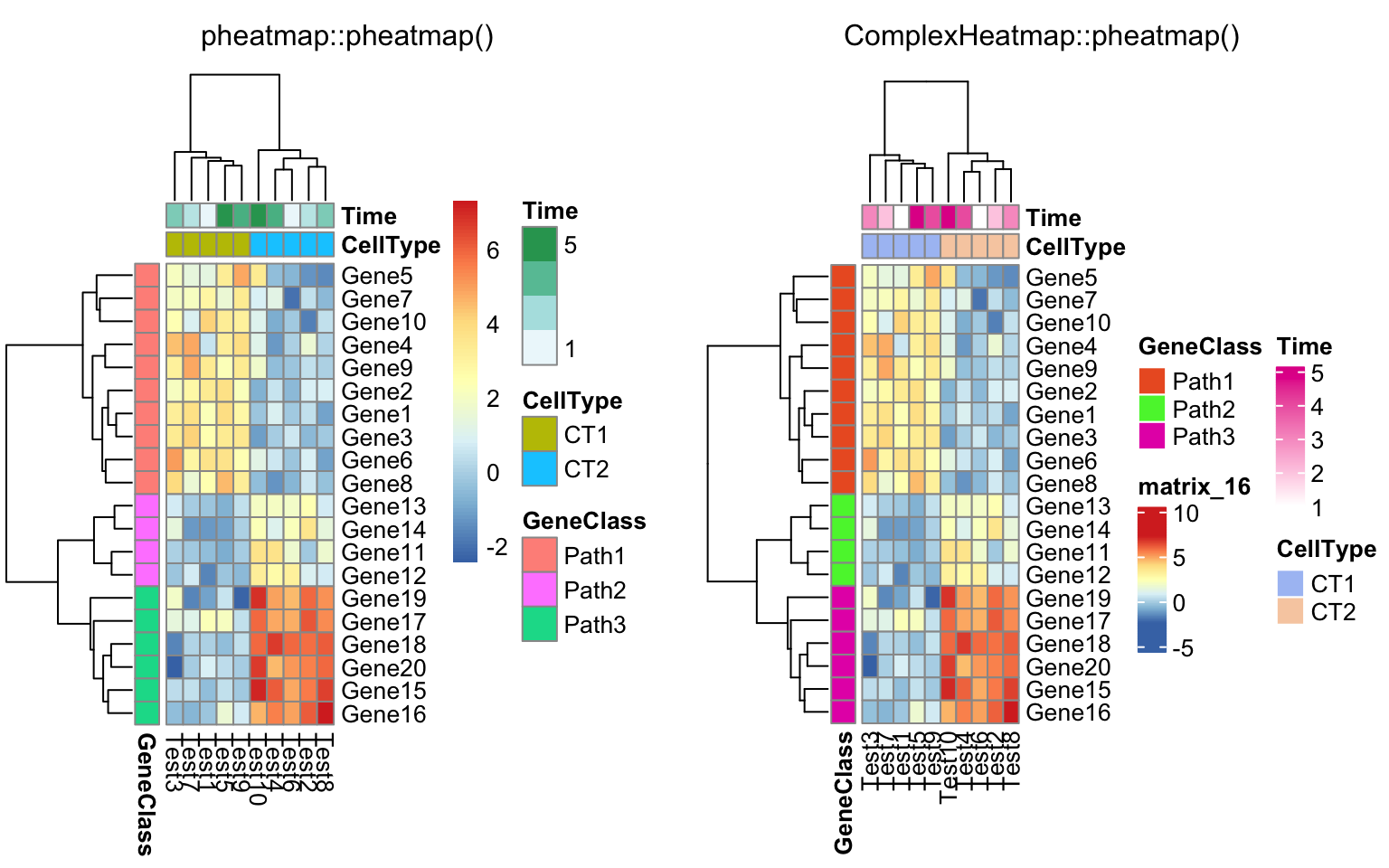compare_pheatmap(test, annotation_col = annotation_col,
annotation_row = annotation_row, angle_col = "45")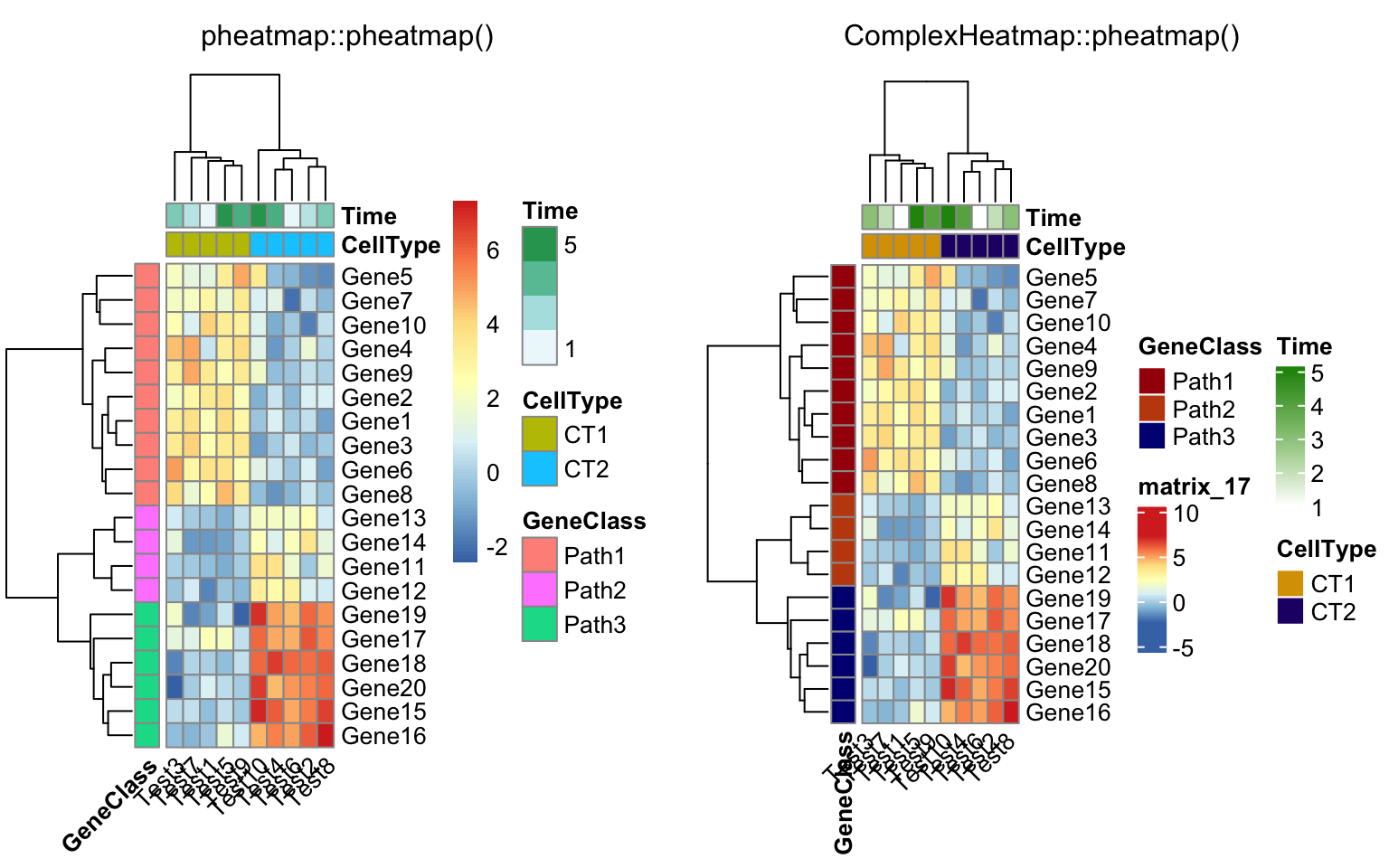compare_pheatmap(test, annotation_col = annotation_col, angle_col = "0")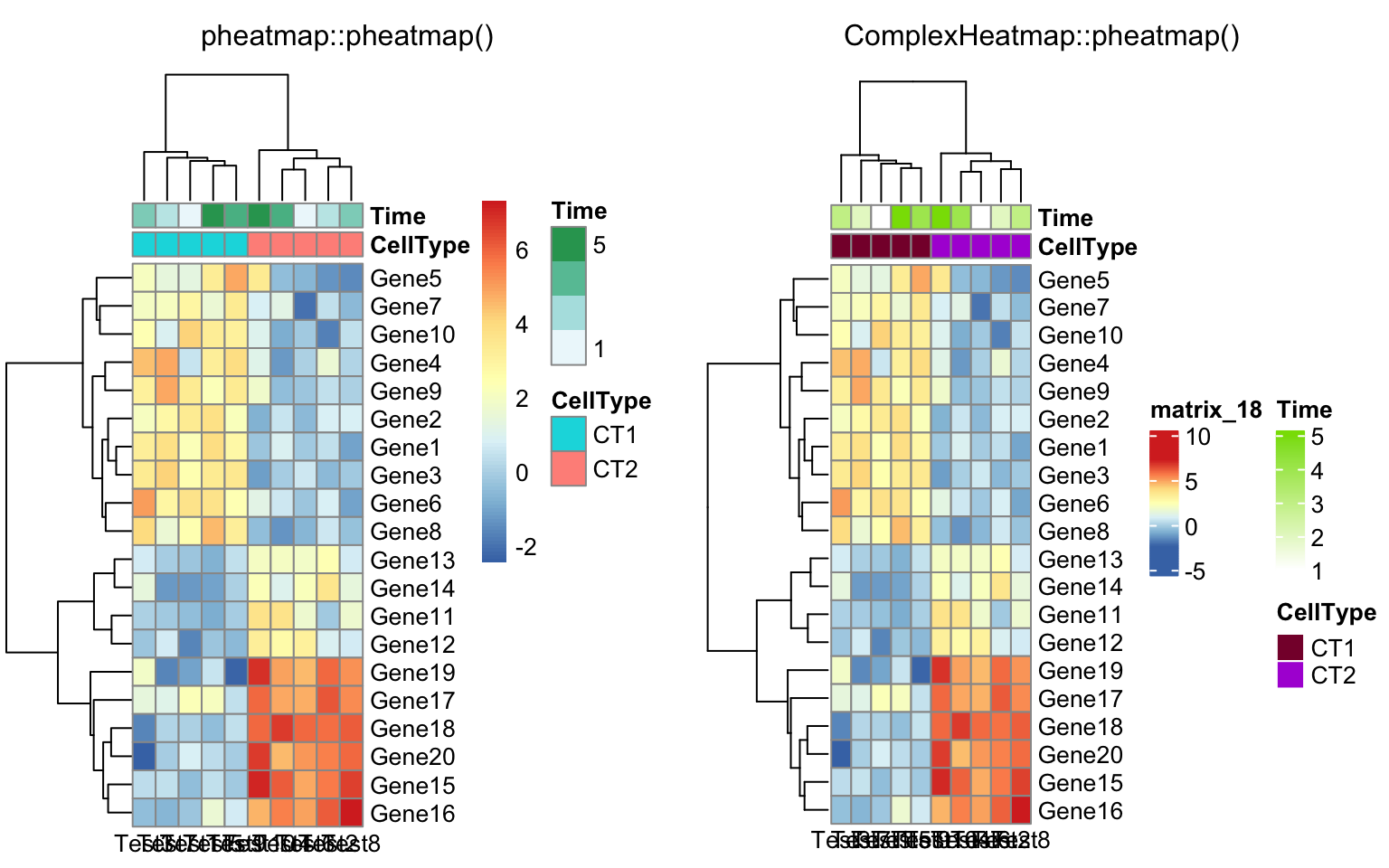ann_colors = list(
Time = c("white", "firebrick"),
CellType = c(CT1 = "#1B9E77", CT2 = "#D95F02"),
GeneClass = c(Path1 = "#7570B3", Path2 = "#E7298A", Path3 = "#66A61E")
)

compare_pheatmap(test, annotation_col = annotation_col,
annotation_colors = ann_colors, main = "Title")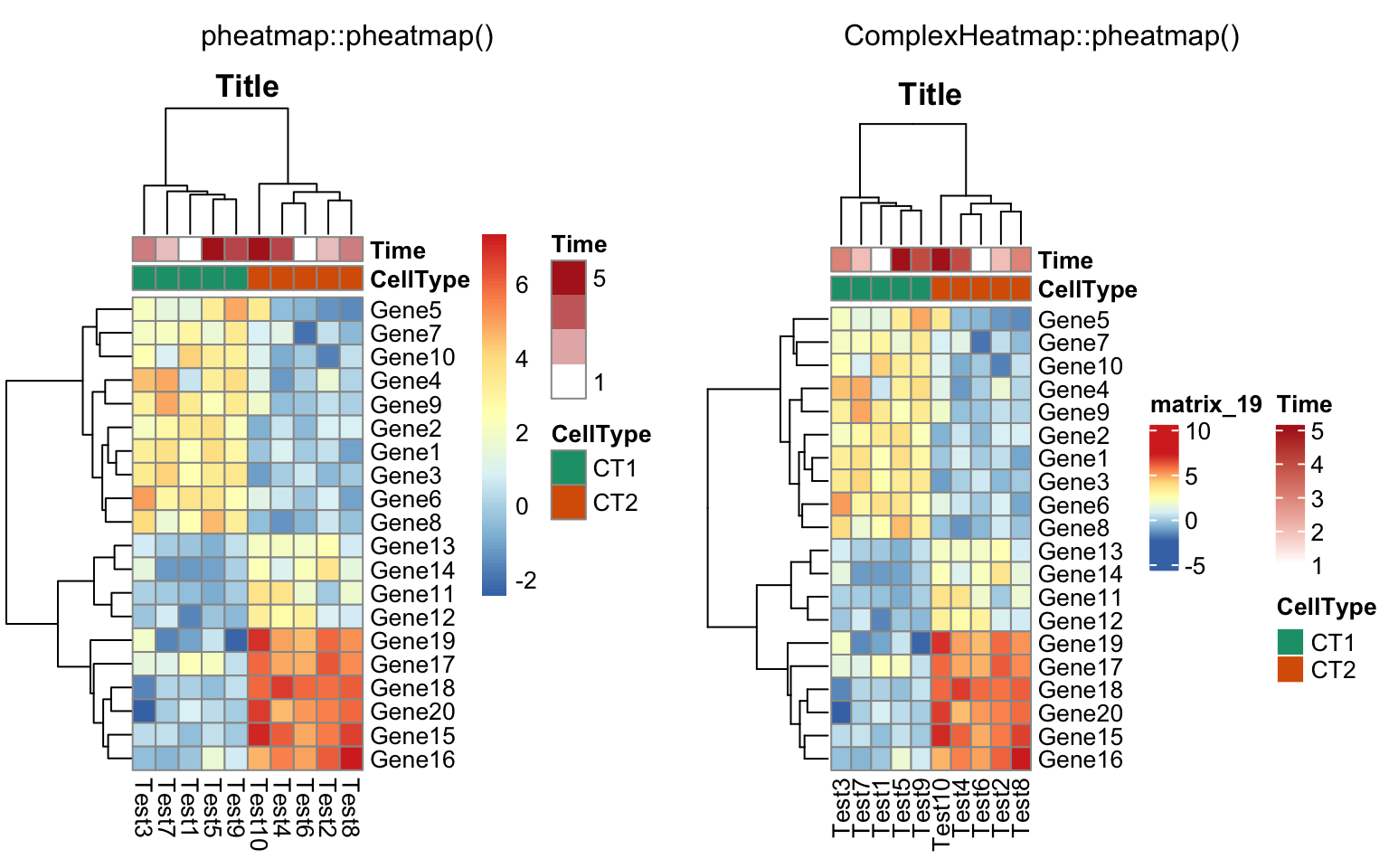compare_pheatmap(test, annotation_col = annotation_col,
annotation_row = annotation_row, annotation_colors = ann_colors)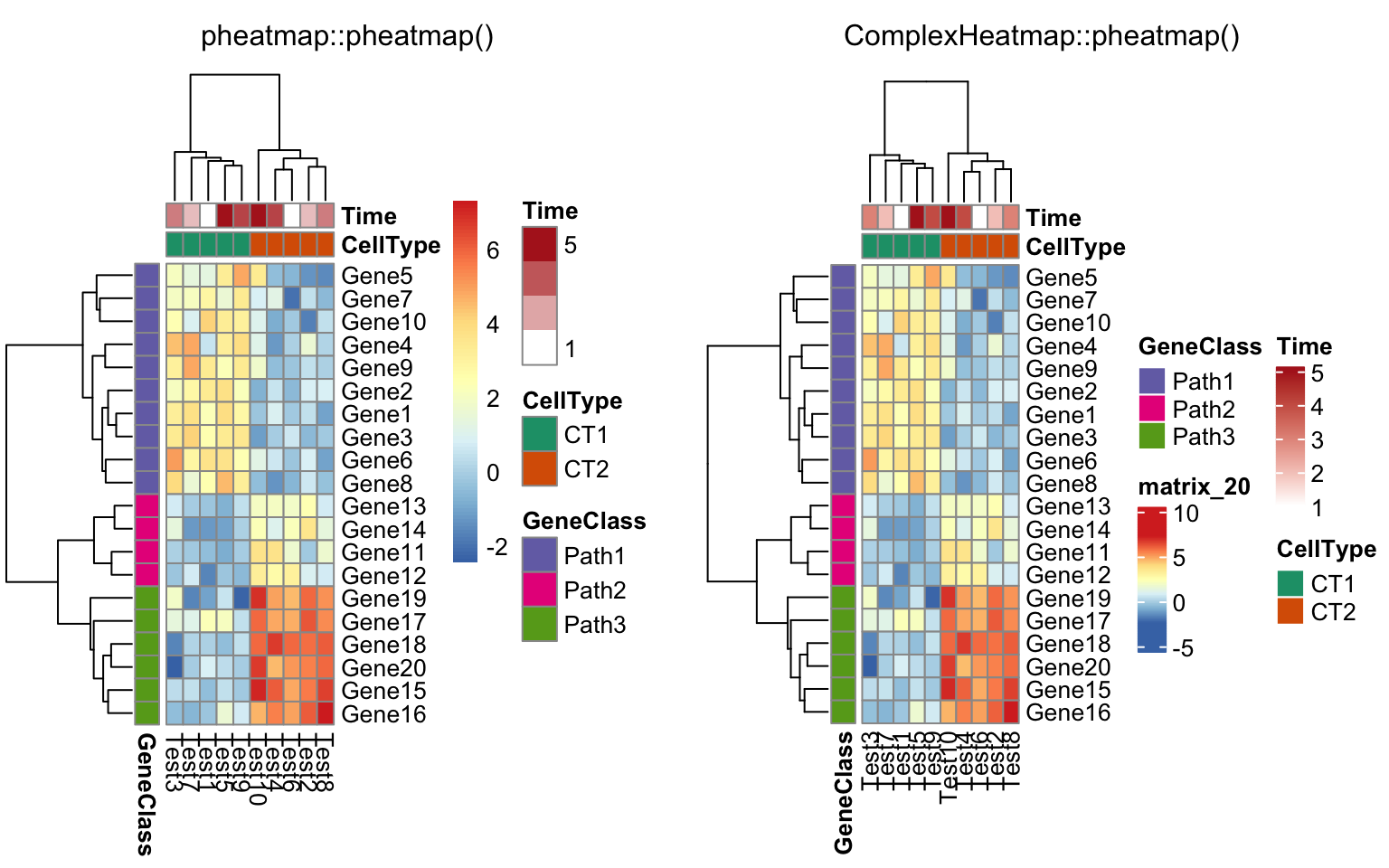compare_pheatmap(test, annotation_col = annotation_col,
annotation_colors = ann_colors)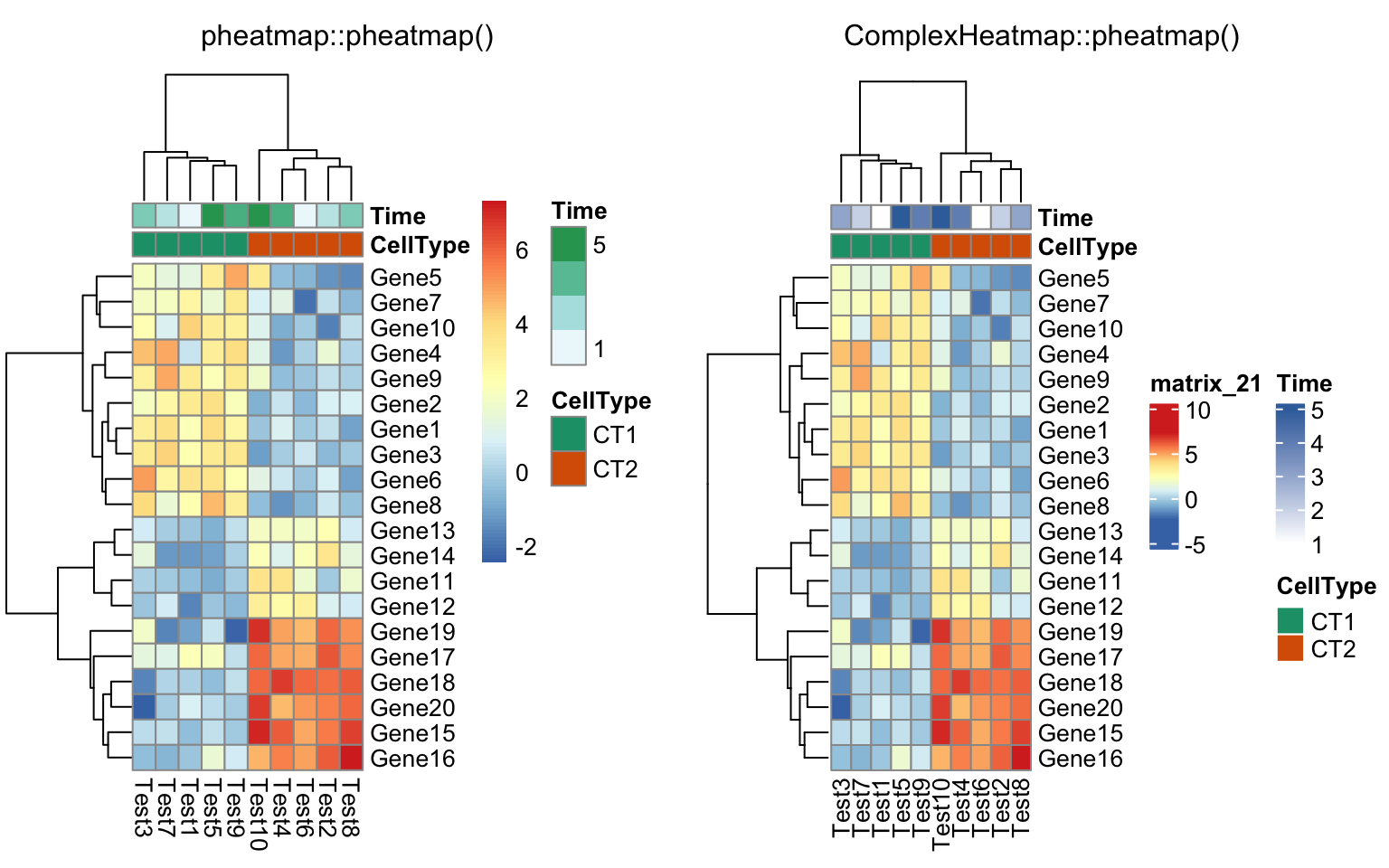compare_pheatmap(test, annotation_col = annotation_col, cluster_rows = FALSE,
gaps_row = c(10, 14))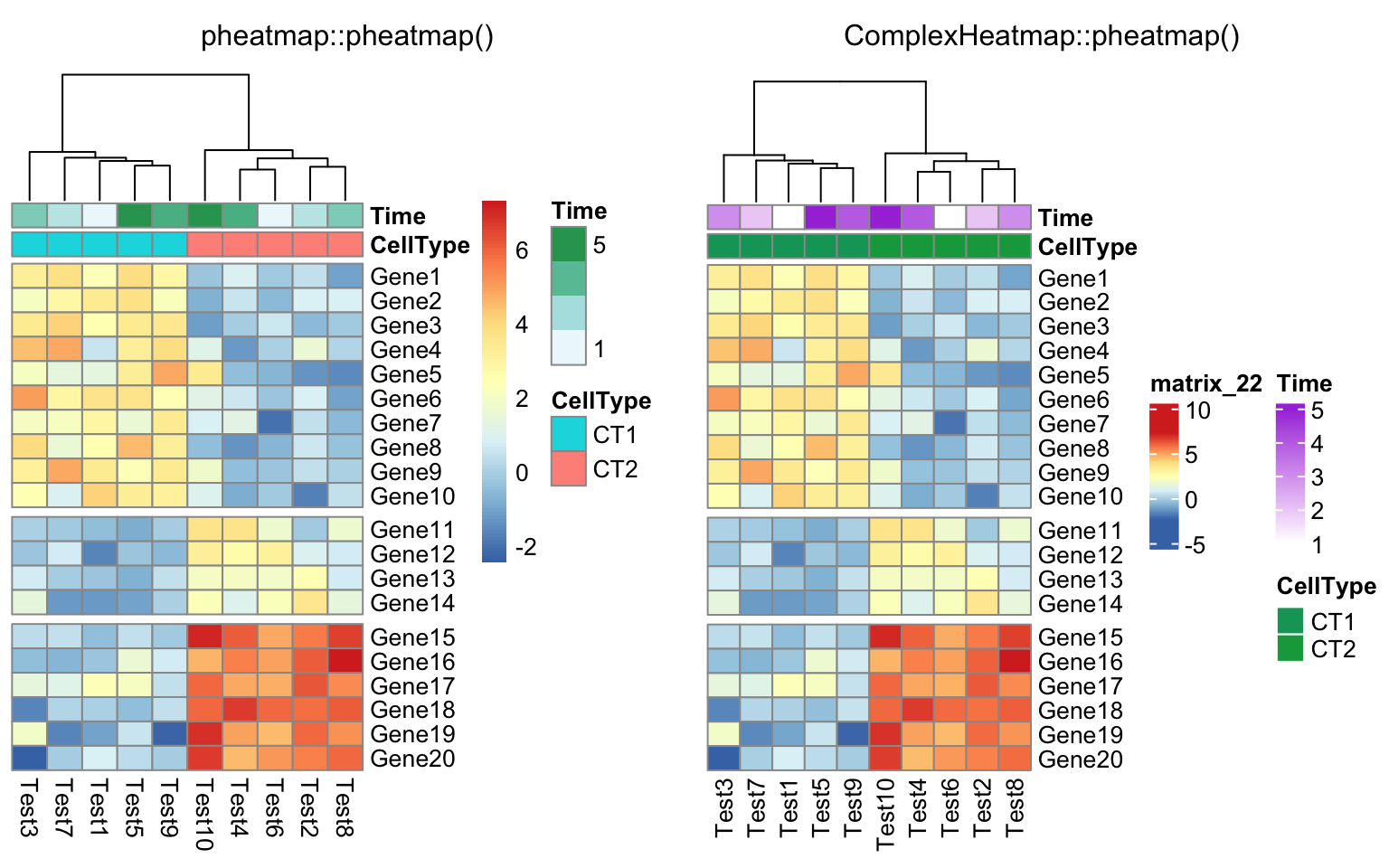compare_pheatmap(test, annotation_col = annotation_col, cluster_rows = FALSE,
gaps_row = c(10, 14), cutree_col = 2)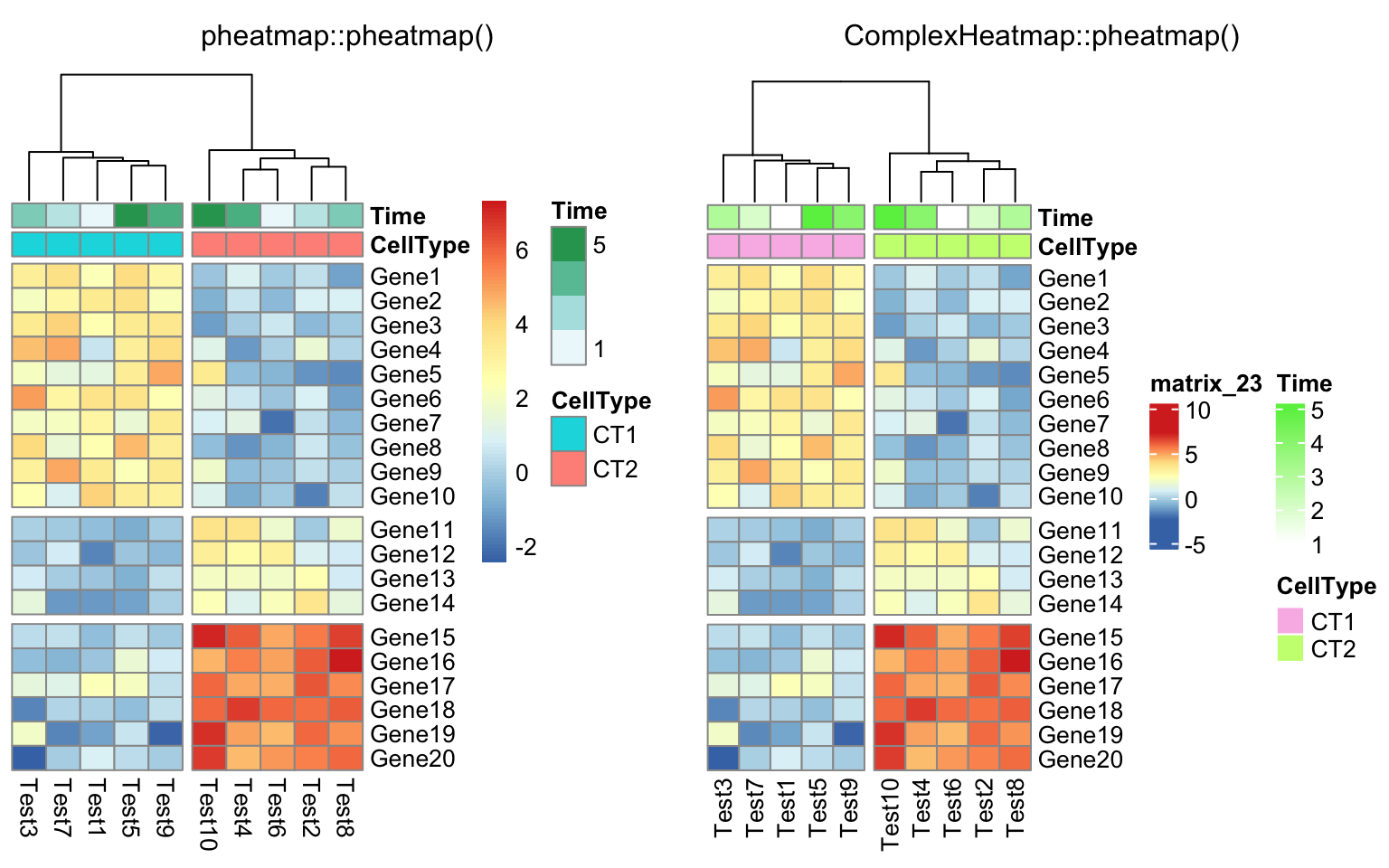labels_row = c("", "", "", "", "", "", "", "", "", "", "", "", "", "", "",
"", "", "Il10", "Il15", "Il1b")
compare_pheatmap(test, annotation_col = annotation_col, labels_row = labels_row)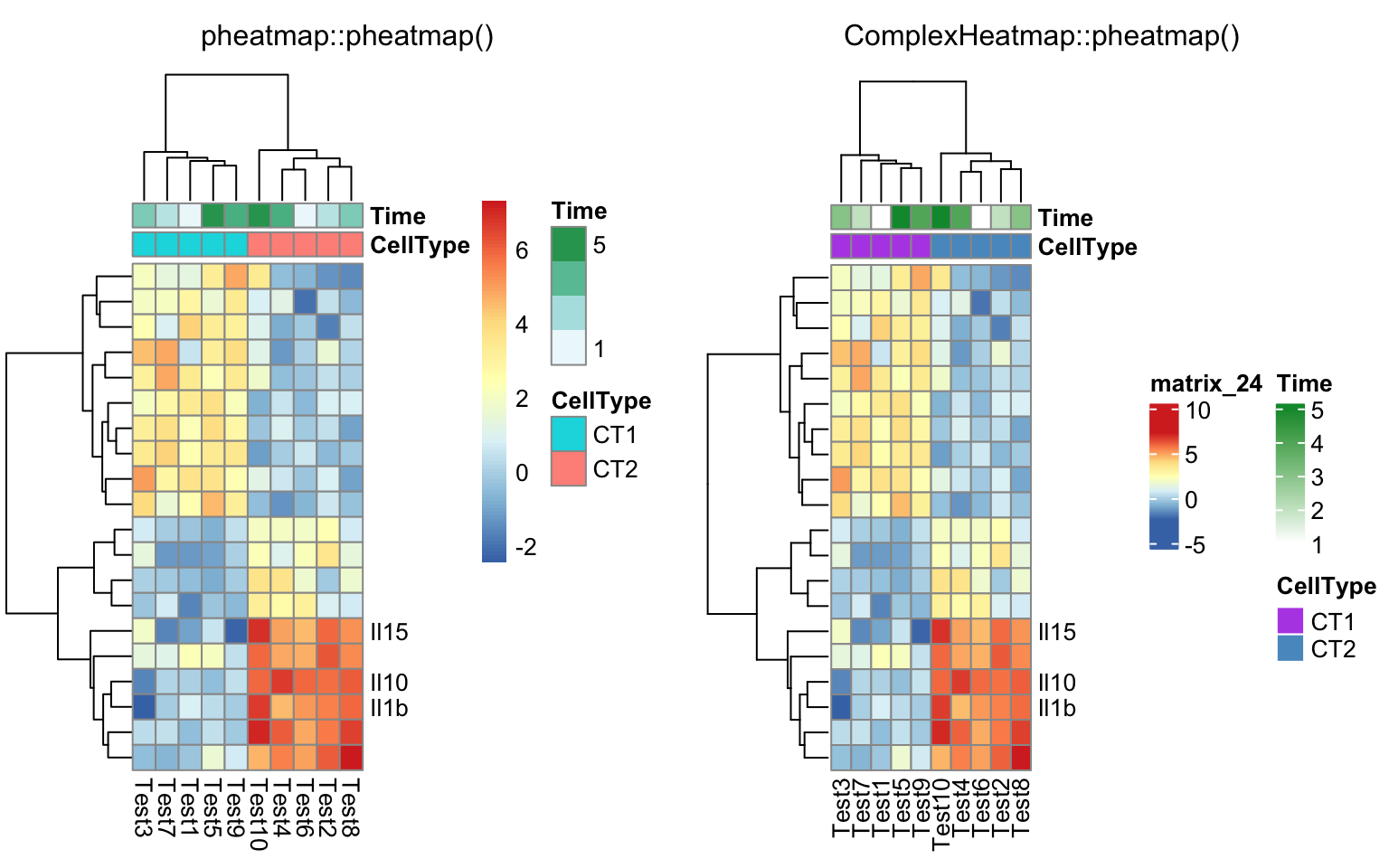drows = dist(test, method = "minkowski")
dcols = dist(t(test), method = "minkowski")
compare_pheatmap(test, clustering_distance_rows = drows,
clustering_distance_cols = dcols)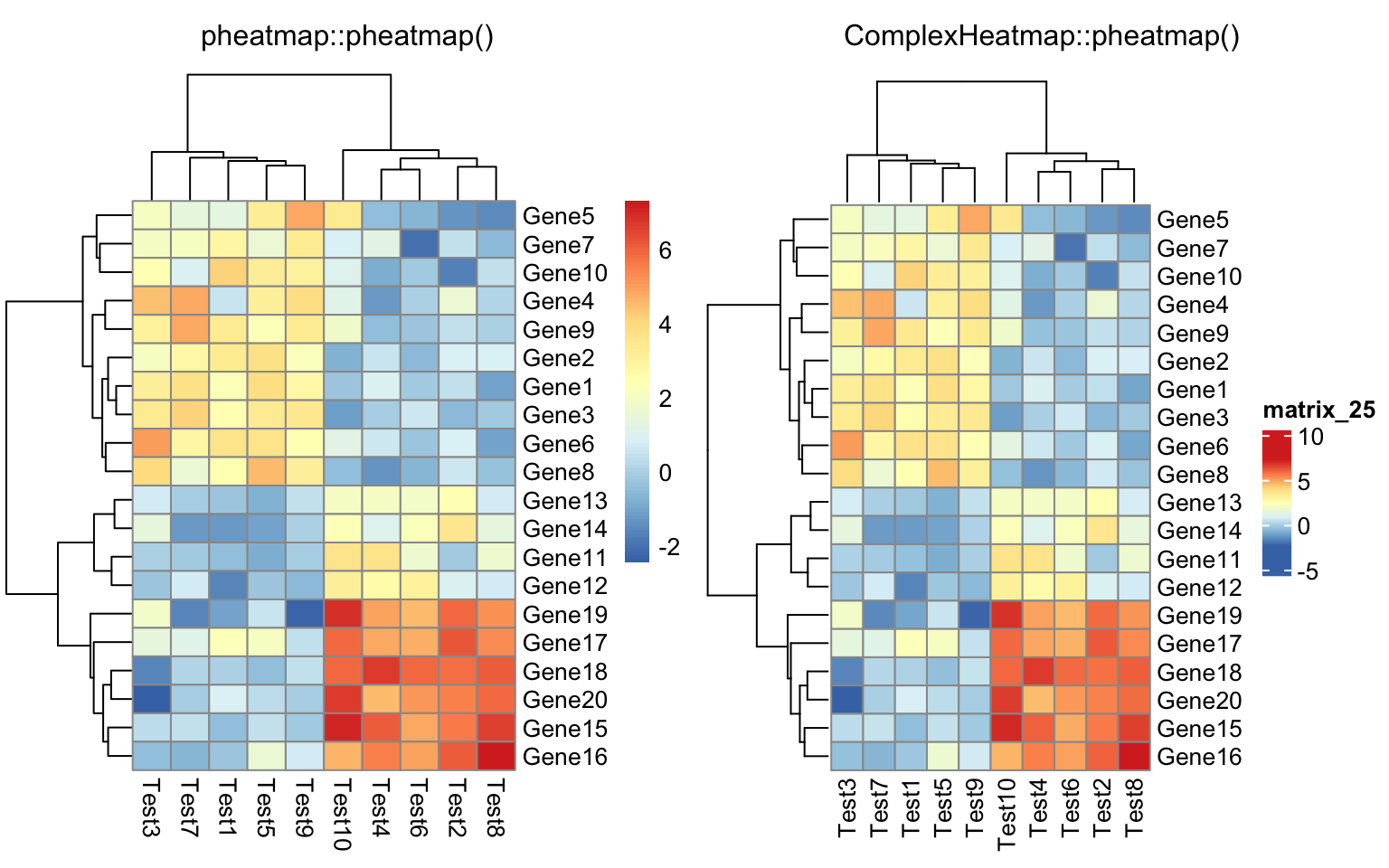library(dendsort)
callback = function(hc, ...){dendsort(hc)}
compare_pheatmap(test, clustering_callback = callback)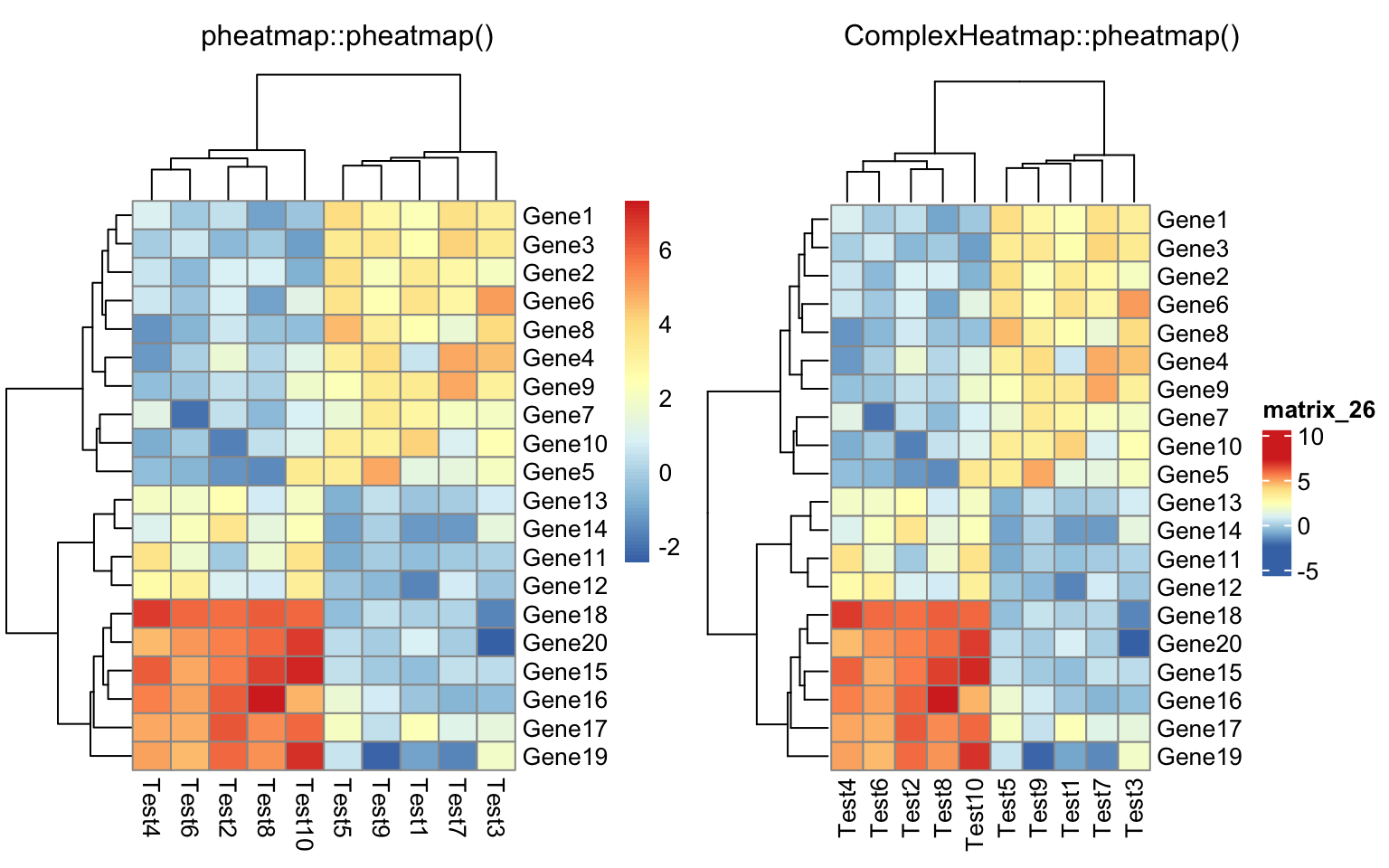## 10.2 cowplot

The cowplot package is used to combine multiple plots into a single figure. In most cases, ComplexHeatmap works perfectly with cowplot, but there are some cases that need special attention.

Also there are some other packages that combine multiple plots, such as multipanelfigure, but I think the mechanism behind is the same.

Following functionalities in ComplexHeatmap cause problems with using cowplot.

1. anno_zoom()/anno_link(): The adjusted positions by these two functions rely on the size of the graphics device.
2. anno_mark(): The same reason as anno_zoom(). The adjusted positions also rely on the device size.
3. When there are too many legends, the legends will be wrapped into multiple columns. The calculation of the legend positions rely on the device size.

In following I demonstrate a case with using the anno_zoom(). Here the example is from the simplifyEnrichment package and the plot shows a GO similarity heatmap with word cloud annotation showing the major biological functions in each group.

You don’t need to really understand the following code. The ht_clusters() function basically draws a heatmap with Heatmap() and add the word cloud annotation by anno_zoom().

library(simplifyEnrichment)
set.seed(1234)
go_id = random_GO(500)
mat = GO_similarity(go_id)
cl = binary_cut(mat)
ht_clusters(mat, cl)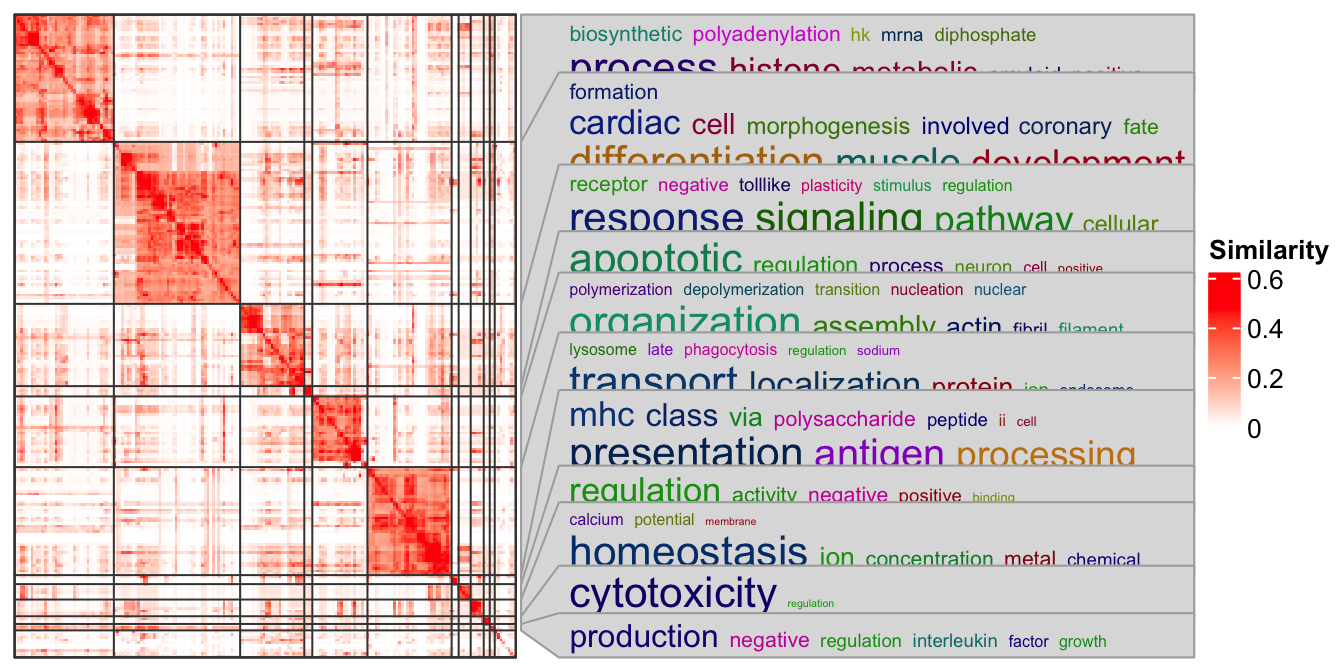Next we put this heatmap as a sub-figure with cowplot. To integrate with cowplot, the heatmap should be captured by grid::grid.grabExpr() as a complex grob object. Note here you need to use draw() function to draw the heatmap explicitly.

library(cowplot)
library(grid)
p1 = rectGrob(width = 0.9, height = 0.9)
p2 = grid.grabExpr(ht_clusters(mat, cl))
p3 = rectGrob(width = 0.9, height = 0.9)

plot_grid(p1,
plot_grid(p2, p3, nrow = 2, rel_heights = c(4, 1)),
nrow = 1, rel_widths = c(1, 9)
)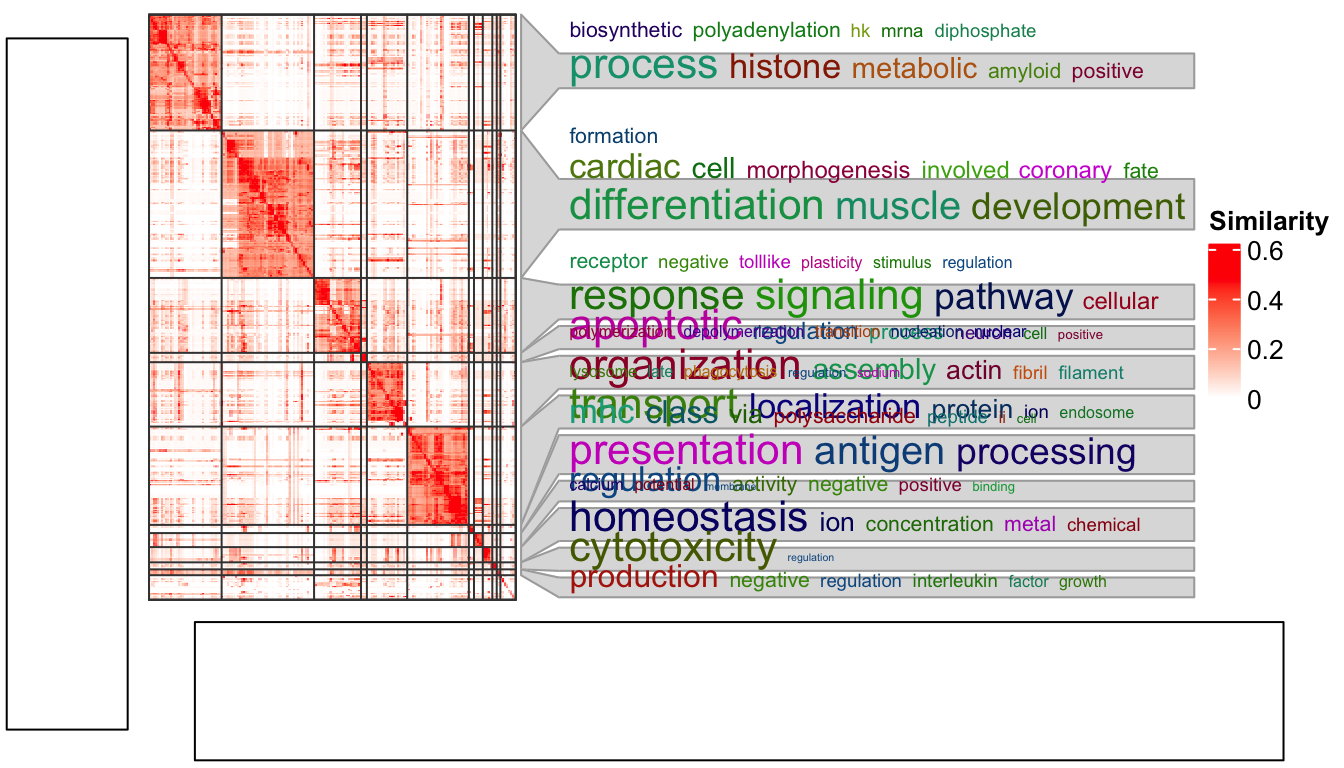Woooo! The word cloud annotation is badly aligned.

There are some details that should be noted for grid.grabExpr() function. It actually opens an invisible graphics device (by pdf(NULL)) with a default size 7x7 inches. Thus, for this line:

p2 = grid.grabExpr(ht_clusters(mat, cl))

The word cloud annotation in p2 is actually calculated in a region of 7x7 inches, and when it is written back to the figure by plot_grid(), the space for p2 changes, that is why the word cloud annotation is wrongly aligned.

On the other hand, if “a simple heatmap” is captured by grid.grabExpr(), e.g.:

p2 = grid.grabExpr(draw(Heatmap(mat)))

when p2 is put back, everything will work fine because now all the heatmap elements are not dependent on the device size and the positions will be automatically adjusted to the new space.

This effect can also be observed by plotting the heatmap in the interactive graphics device and resizing the window by dragging it.

The solution is rather simple. Since the reason for this inconsistency is the different space between where it is captured and where it is drawn, we only need to capture the heatmap under the device with the same size as where it is going to be put.

As in the layout which we set in the plot_grid() function, the heatmap occupies 9/10 width and 4/5 height of the figure. So, the width and height of the space for the heatmap is calculated as follows and assigned to the width and height arguments in grid.grabExpr().

w = convertWidth(unit(1, "npc")*(9/10), "inch", valueOnly = TRUE)
h = convertHeight(unit(1, "npc")*(4/5), "inch", valueOnly = TRUE)
p2 = grid.grabExpr(ht_clusters(mat, cl), width = w, height = h)

plot_grid(p1,
plot_grid(p2, p3, nrow = 2, rel_heights = c(4, 1)),
nrow = 1, rel_widths = c(1, 9)
)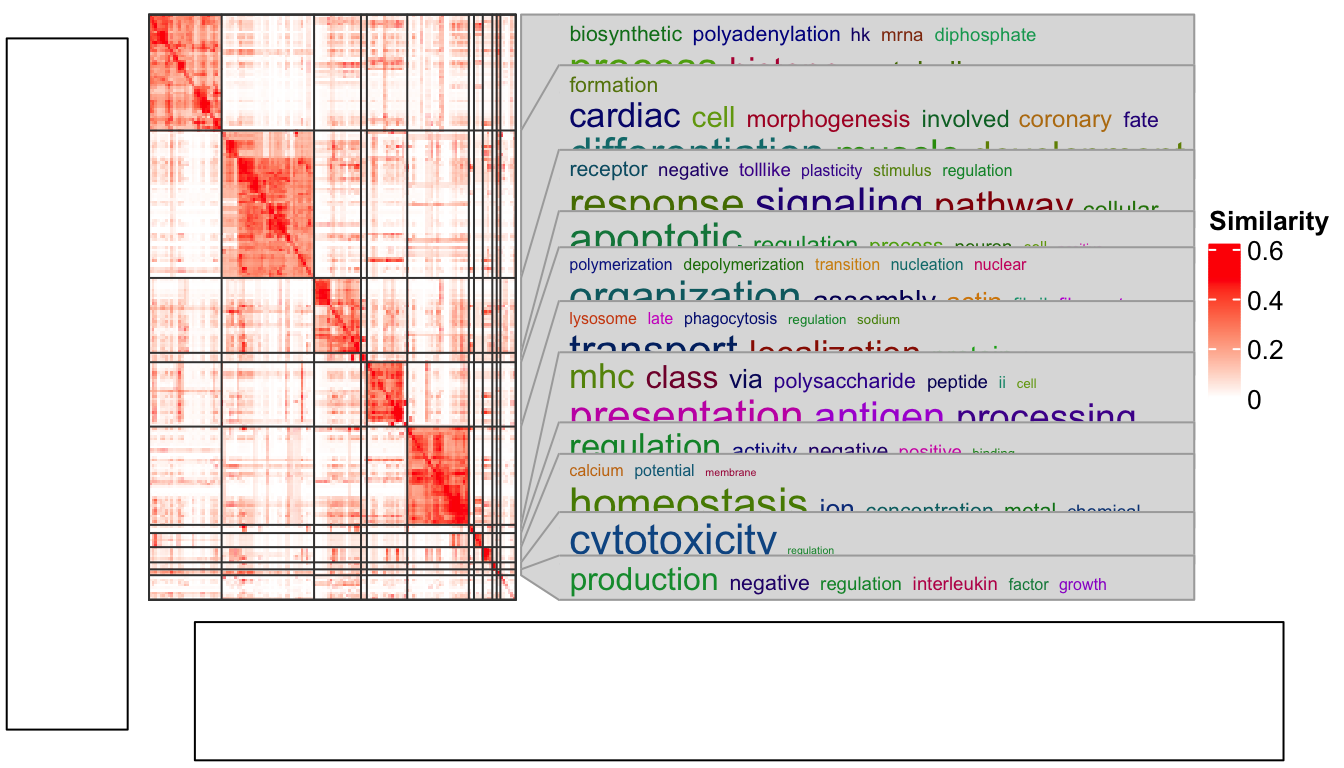Now everthing is back to normal!

## 10.3 gridtext

The gridtext package provides a nice and easy way for rendering text under the grid system. From version 2.3.3 of ComplexHeatmap, text-related elements can be rendered by gridtext.

For all text-related elements, the text needs to be wrapped by gt_render() function, which marks the text and adds related parameters that are going to be processed by gridtext.

Currently ComplexHeatmap supports gridtext::richtext_grob(), so some of the parameters for richtext_grob() can be passed via gt_render().

gt_render("foo", r = unit(2, "pt"), padding = unit(c(2, 2, 2, 2), "pt"))
##  "foo"
## attr(,"class")
##  "gridtext"
## attr(,"param")
## attr(,"param")$r ##  2points ## ## attr(,"param")$padding
##  2points 2points 2points 2points

For each heatmap element, e.g. column title, graphic parameters can be set by the companion argument, e.g. column_title_gp. To make it simpler, all graphic parameters set by box_gp are merged with *_gp by adding box_ prefix, e.g.:

..., column_title = gt_render("foo"), column_title_gp = gpar(col = "red", box_fill = "blue"), ...

Graphic parameters can also be specified inside gt_render(). Following is the same as the one above:

..., column_title = gt_render("foo", gp = gpar(col = "red", box_fill = "blue")), ...

### 10.3.1 Titles

set.seed(123)
mat = matrix(rnorm(100), 10)
rownames(mat) = letters[1:10]
Heatmap(mat,
column_title = gt_render("Some <span style='color:blue'>blue text **in bold.**</span><br>And *italics text.*<br>And some <span style='font-size:18pt; color:black'>large</span> text.",
r = unit(2, "pt"),
padding = unit(c(2, 2, 2, 2), "pt")),
column_title_gp = gpar(box_fill = "orange"))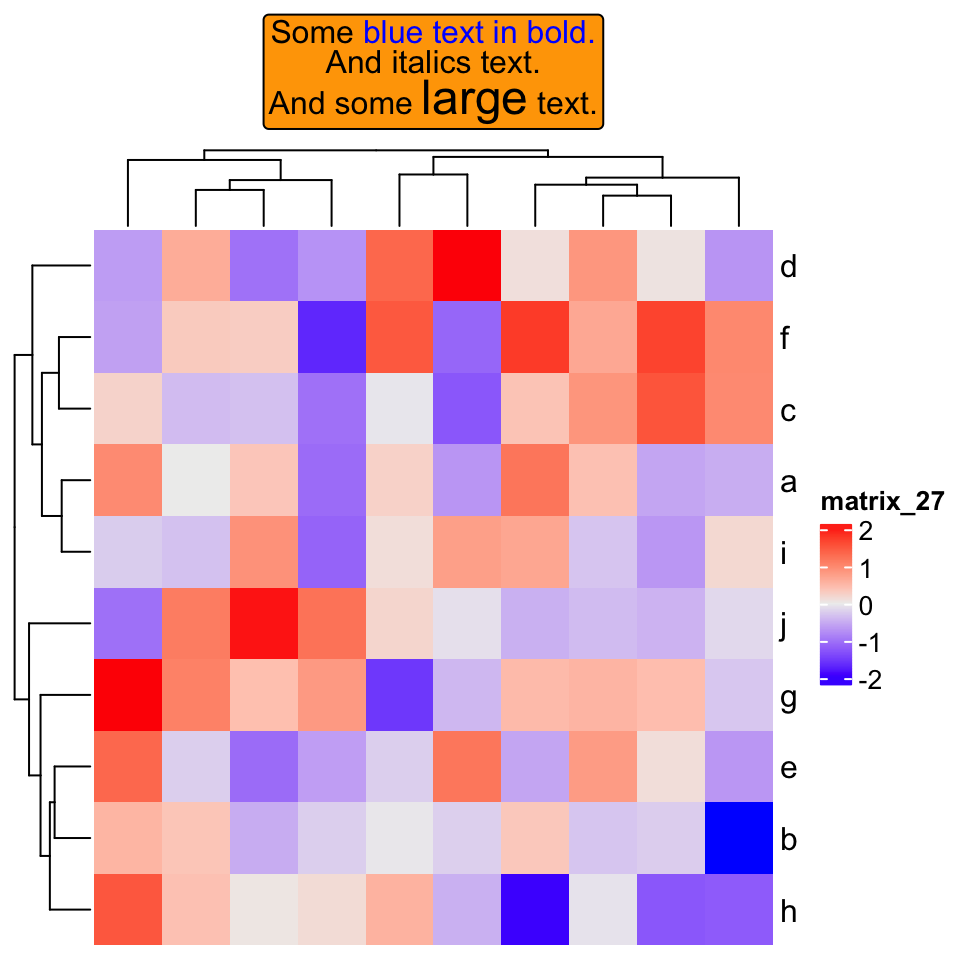If heatmap is split:

Heatmap(mat,
row_km = 2,
row_title = gt_render(c("**title1**", "_title2_")),
row_title_gp = gpar(box_fill = c("yellow", "blue")))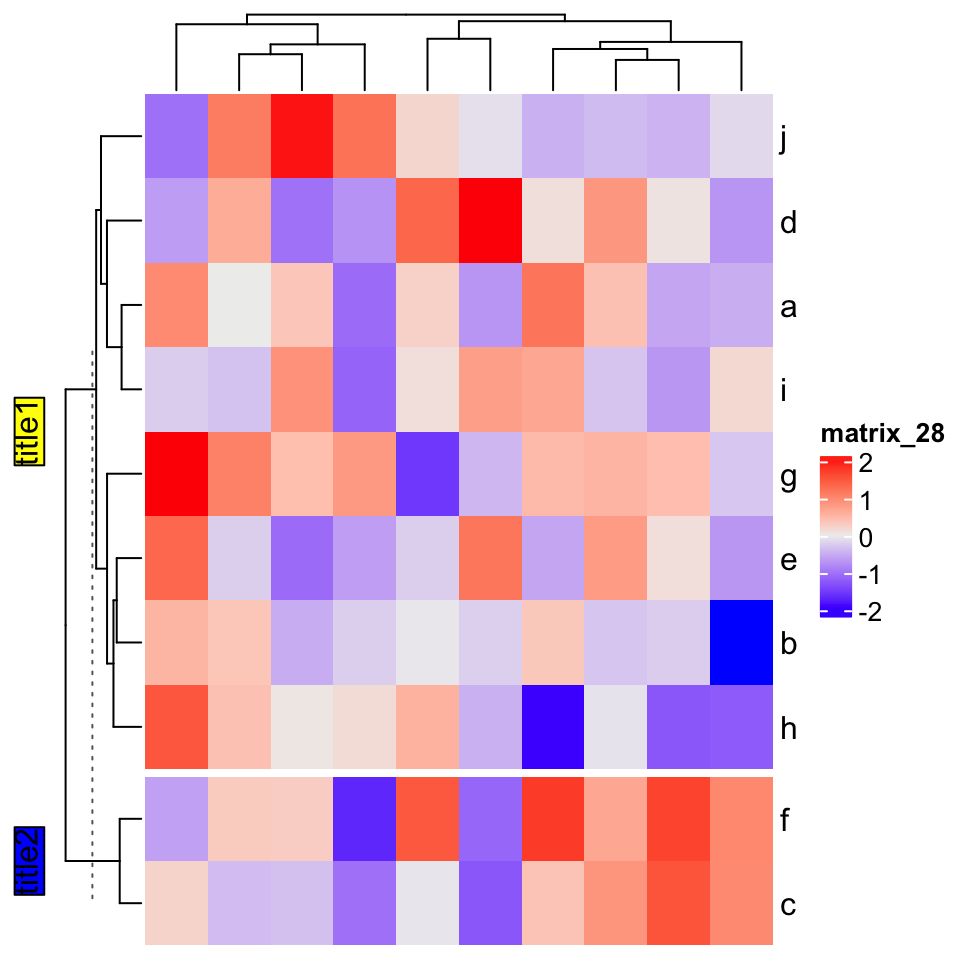### 10.3.2 Row/column names

Rendered row/column names should be explicitly specified by row_labels/column_labels

Heatmap(mat,
row_labels = gt_render(letters[1:10], padding = unit(c(2, 10, 2, 10), "pt")),
row_names_gp = gpar(box_col = rep(c("red", "green"), times = 5)))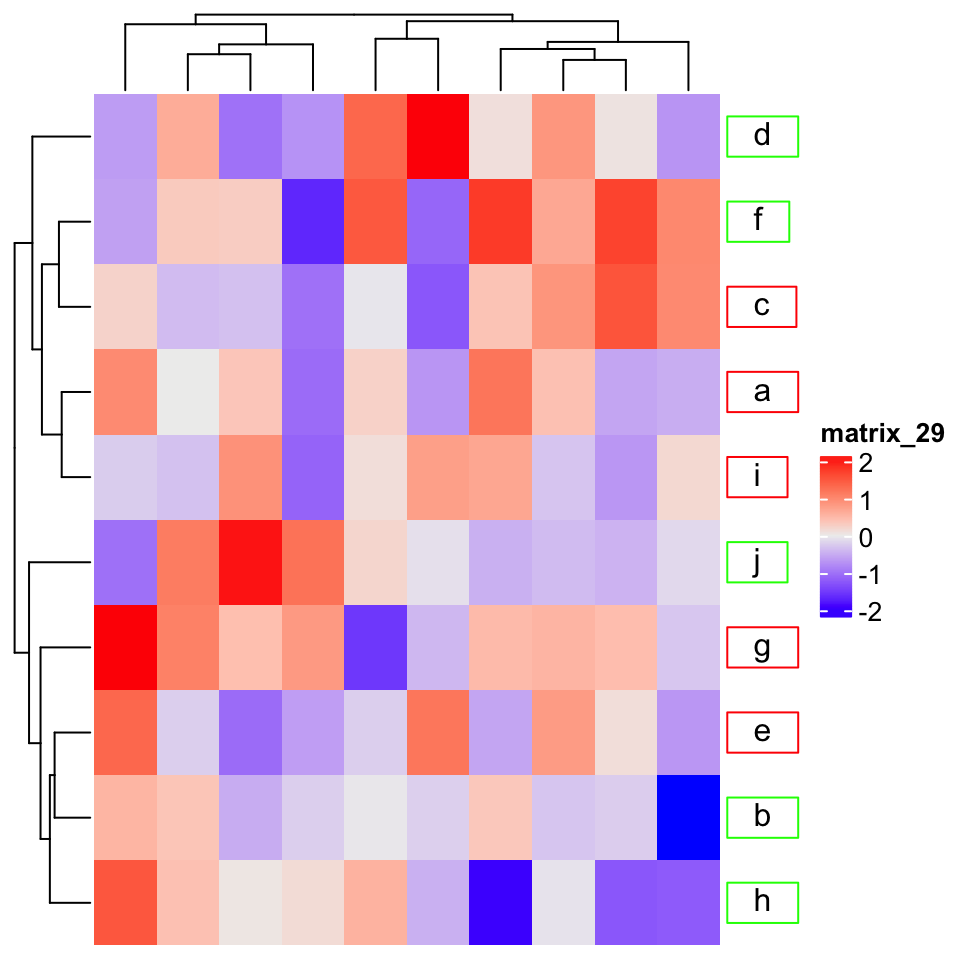### 10.3.3 Annotation labels

annotation_label argument should be as rendered text.

ha = HeatmapAnnotation(foo = letters[1:10],
annotation_label = gt_render("**Annotation** _one_",
gp = gpar(box_col = "black")),
show_legend = FALSE)
Heatmap(mat, top_annotation = ha)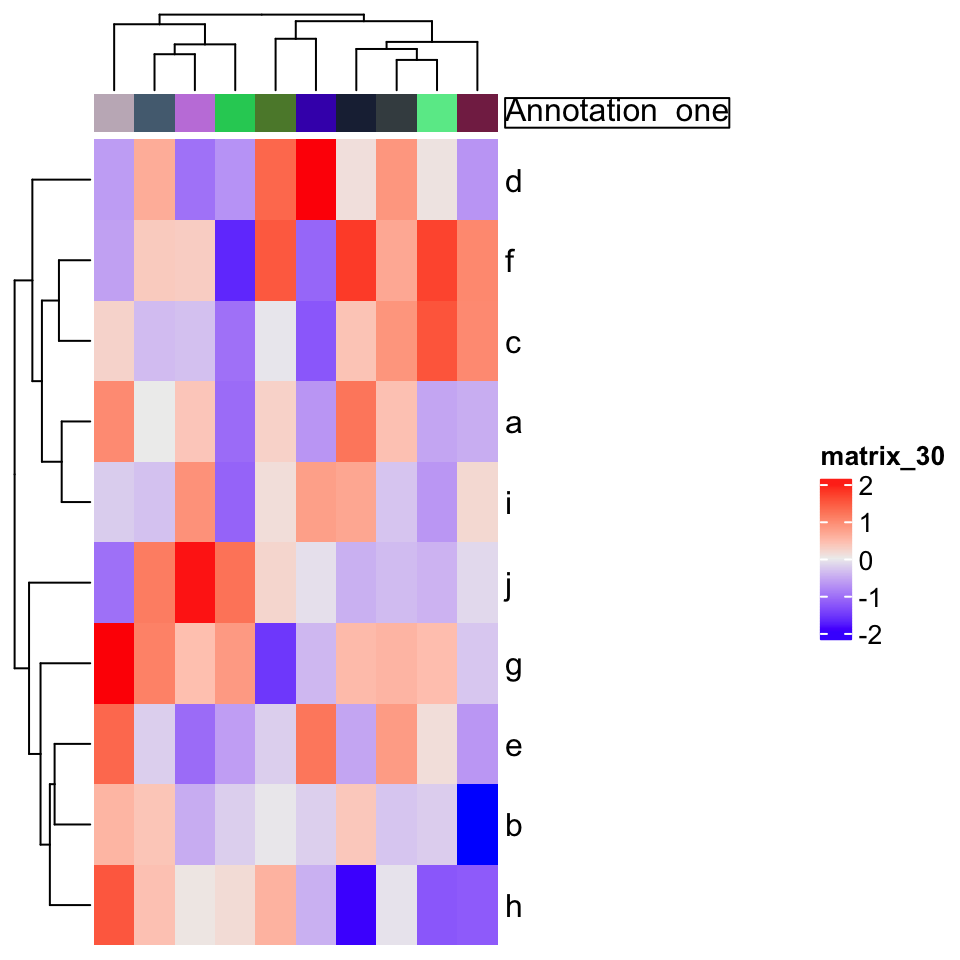### 10.3.4 Text annotation

rowAnnotation(
foo = anno_text(gt_render(sapply(LETTERS[1:10], strrep, 10), align_widths = TRUE),
gp = gpar(box_col = "blue", box_lwd = 2),
just = "right",
location = unit(1, "npc")
)) + Heatmap(mat)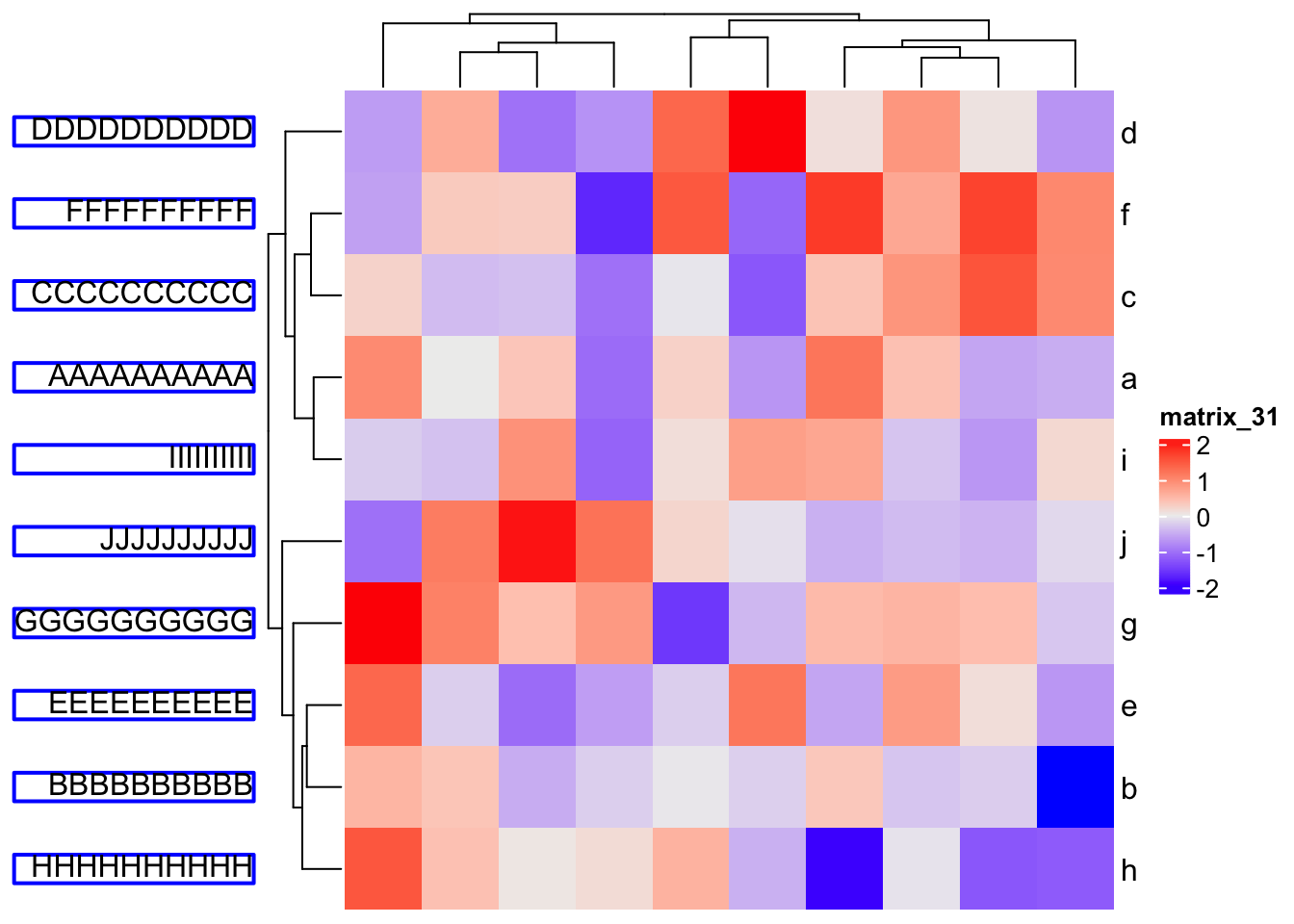### 10.3.5 Legend

Heatmap(mat,
heatmap_legend_param = list(
title = gt_render("<span style='color:orange'>**Legend title**</span>"),
title_gp = gpar(box_fill = "grey"),
at = c(-3, 0, 3),
labels = gt_render(c("*negative* three", "zero", "*positive* three"))
))